Скачать презентацию maths project work chapter 8 comparing

59a9aec619d5d9d68f0acbe791e08e2a.ppt

• Количество слайдов: 92maths project workchapter : 8 comparing quantities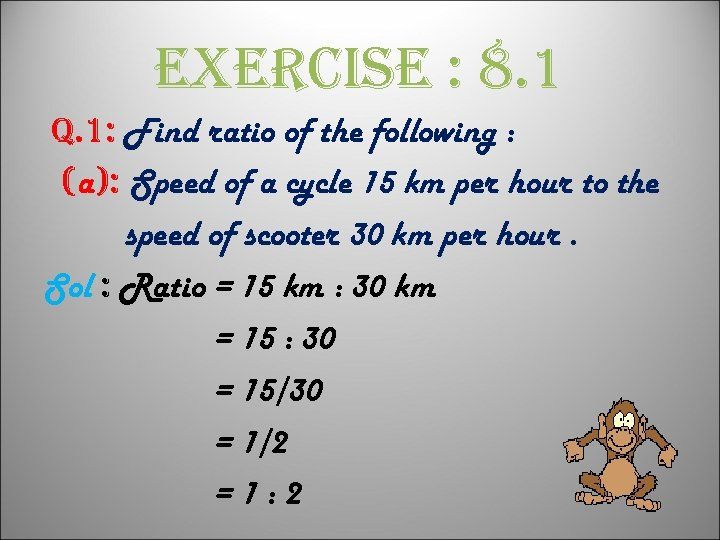exercise : 8. 1 q. 1: Find ratio of the following : (a): Speed of a cycle 15 km per hour to the speed of scooter 30 km per hour. Sol : Ratio = 15 km : 30 km = 15 : 30 = 15/30 = 1/2 =1: 2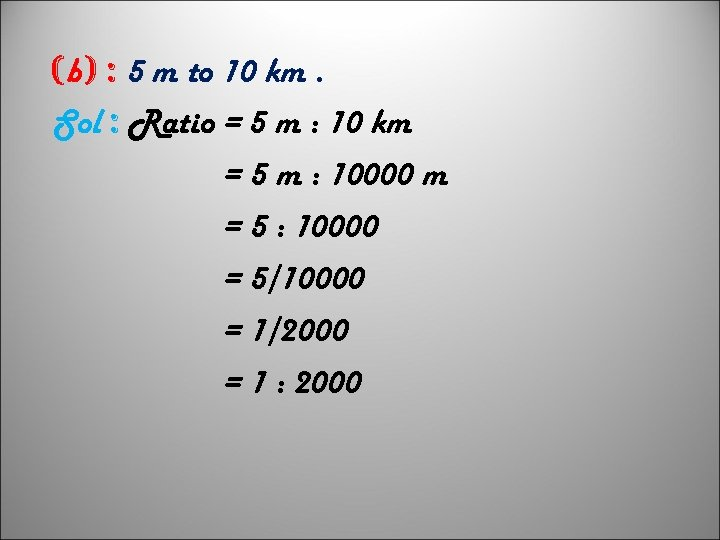(b) : 5 m to 10 km. Sol : Ratio = 5 m : 10 km = 5 m : 10000 m = 5 : 10000 = 5/10000 = 1/2000 = 1 : 2000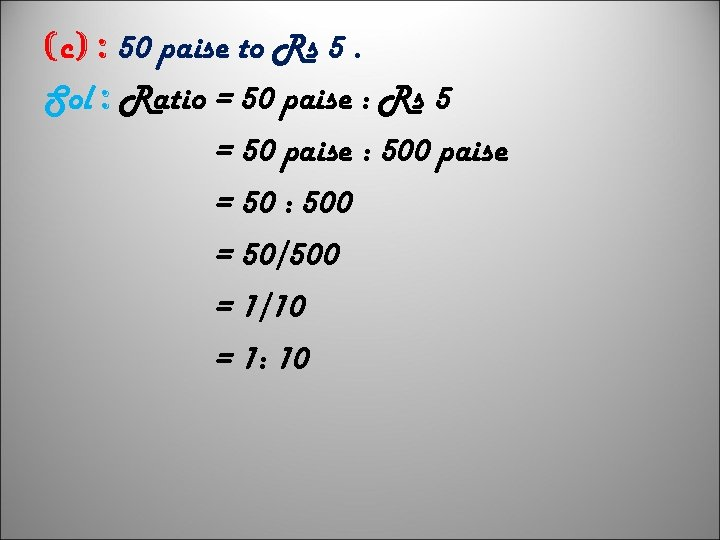(c) : 50 paise to Rs 5. Sol : Ratio = 50 paise : Rs 5 = 50 paise : 500 paise = 50 : 500 = 50/500 = 1/10 = 1: 10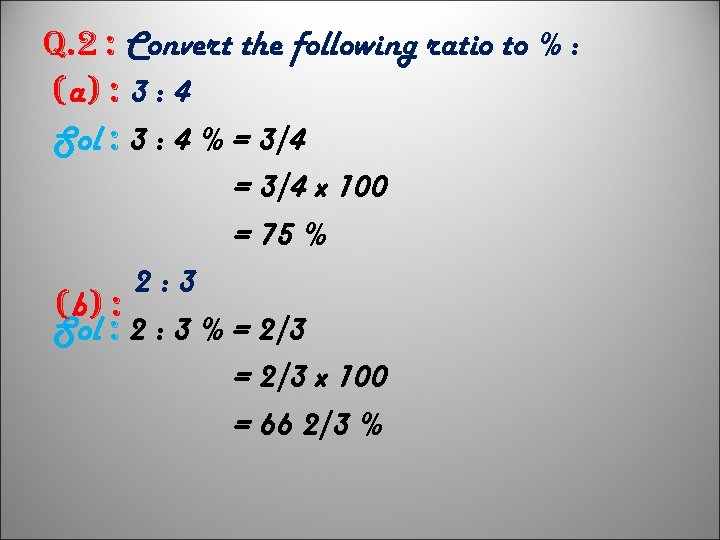q. 2 : Convert the following ratio to % : (a) : 3 : 4 Sol : 3 : 4 % = 3/4 x 100 = 75 % 2: 3 (b) : Sol : 2 : 3 % = 2/3 x 100 = 66 2/3 %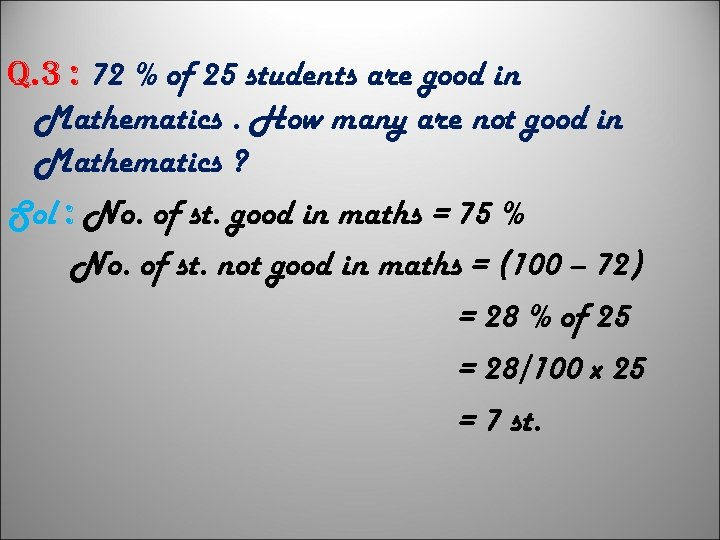q. 3 : 72 % of 25 students are good in Mathematics. How many are not good in Mathematics ? Sol : No. of st. good in maths = 75 % No. of st. not good in maths = (100 – 72) = 28 % of 25 = 28/100 x 25 = 7 st.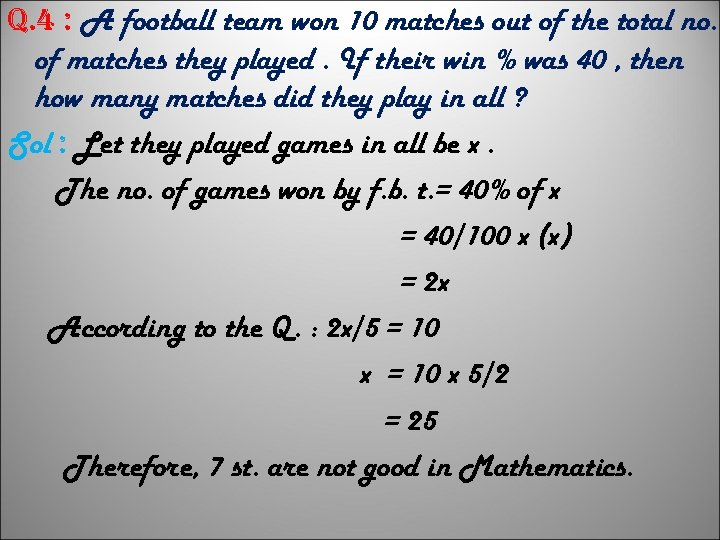q. 4 : A football team won 10 matches out of the total no. of matches they played. If their win % was 40 , then how many matches did they play in all ? Sol : Let they played games in all be x. The no. of games won by f. b. t. = 40% of x = 40/100 x (x) = 2 x According to the Q. : 2 x/5 = 10 x 5/2 = 25 Therefore, 7 st. are not good in Mathematics.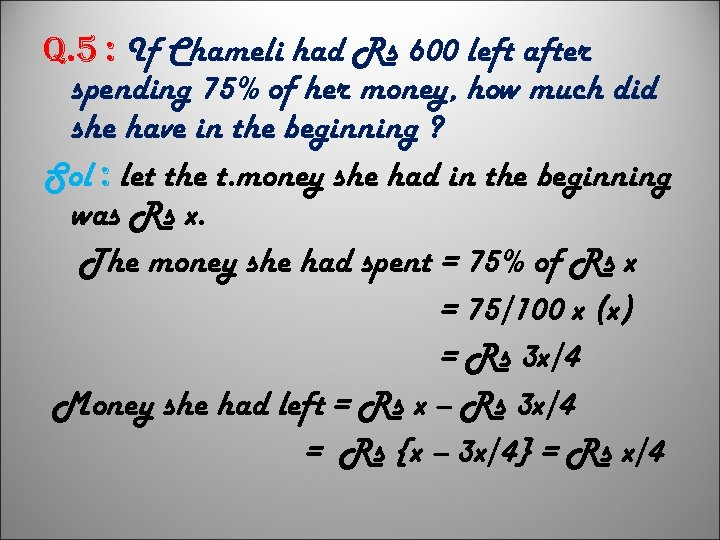q. 5 : If Chameli had Rs 600 left after spending 75% of her money, how much did she have in the beginning ? Sol : let the t. money she had in the beginning was Rs x. The money she had spent = 75% of Rs x = 75/100 x (x) = Rs 3 x/4 Money she had left = Rs x – Rs 3 x/4 = Rs {x – 3 x/4} = Rs x/4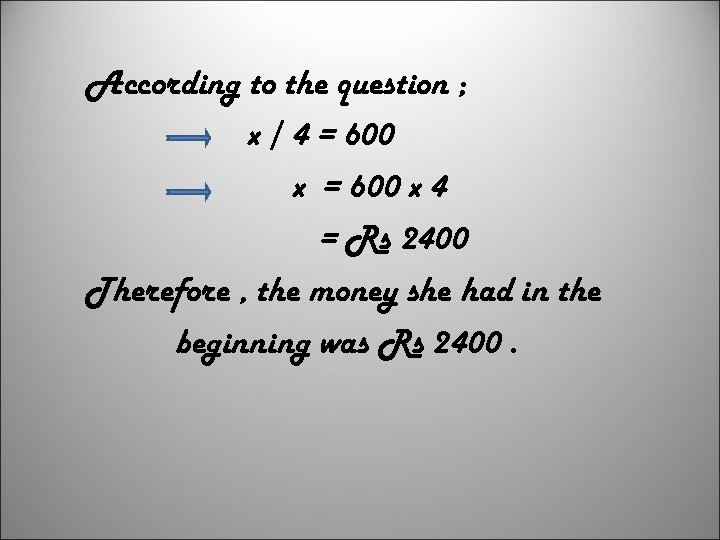According to the question ; x / 4 = 600 x 4 = Rs 2400 Therefore , the money she had in the beginning was Rs 2400.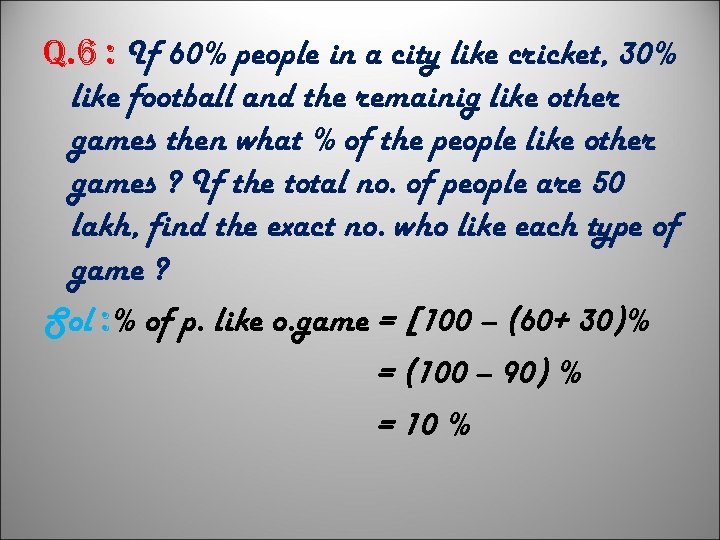q. 6 : If 60% people in a city like cricket, 30% like football and the remainig like other games then what % of the people like other games ? If the total no. of people are 50 lakh, find the exact no. who like each type of game ? Sol : % of p. like o. game = [100 – (60+ 30)% = (100 – 90) % = 10 %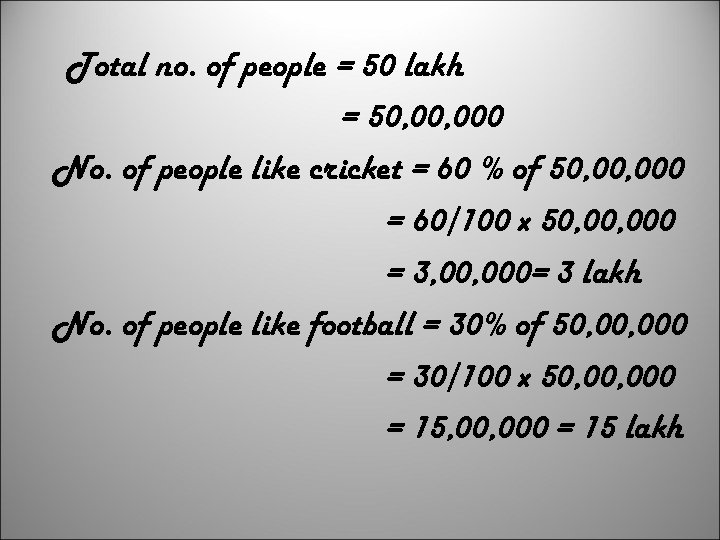Total no. of people = 50 lakh = 50, 000 No. of people like cricket = 60 % of 50, 000 = 60/100 x 50, 000 = 3, 000= 3 lakh No. of people like football = 30% of 50, 000 = 30/100 x 50, 000 = 15, 000 = 15 lakh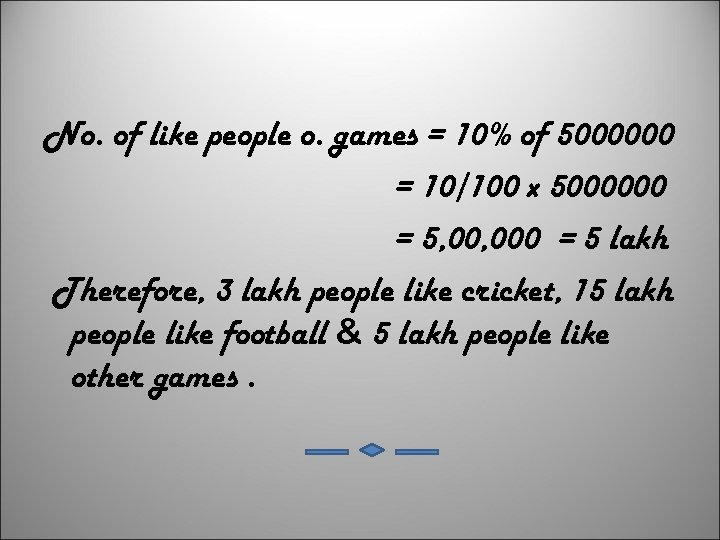No. of like people o. games = 10% of 5000000 = 10/100 x 5000000 = 5, 000 = 5 lakh Therefore, 3 lakh people like cricket, 15 lakh people like football & 5 lakh people like other games.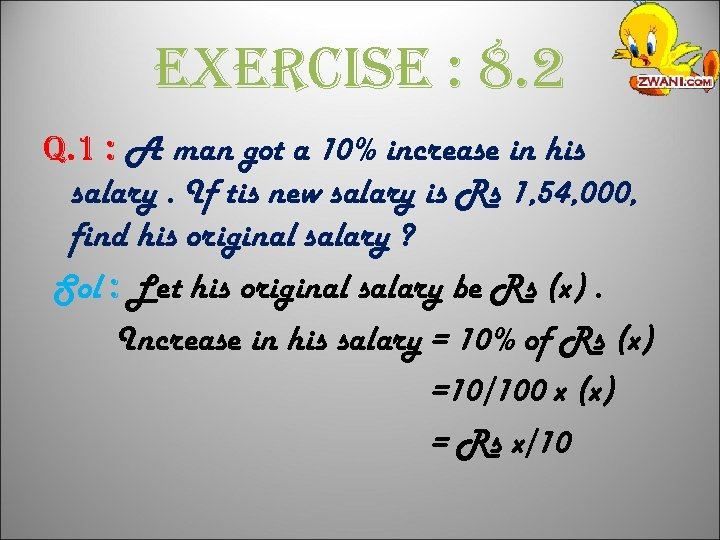exercise : 8. 2 q. 1 : A man got a 10% increase in his salary. If tis new salary is Rs 1, 54, 000, find his original salary ? Sol : Let his original salary be Rs (x). Increase in his salary = 10% of Rs (x) =10/100 x (x) = Rs x/10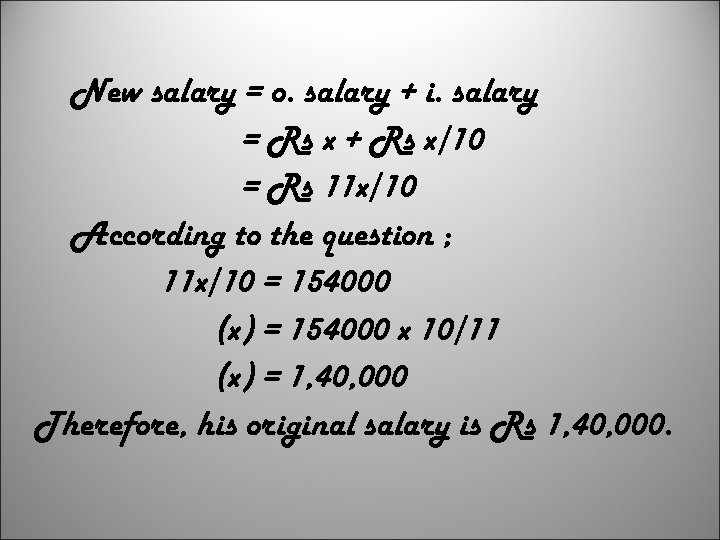New salary = o. salary + i. salary = Rs x + Rs x/10 = Rs 11 x/10 According to the question ; 11 x/10 = 154000 (x) = 154000 x 10/11 (x) = 1, 40, 000 Therefore, his original salary is Rs 1, 40, 000.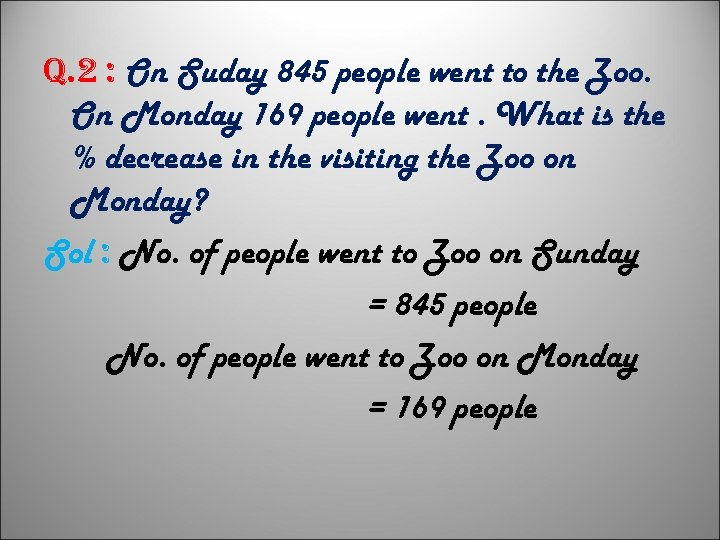q. 2 : On Suday 845 people went to the Zoo. On Monday 169 people went. What is the % decrease in the visiting the Zoo on Monday? Sol : No. of people went to Zoo on Sunday = 845 people No. of people went to Zoo on Monday = 169 people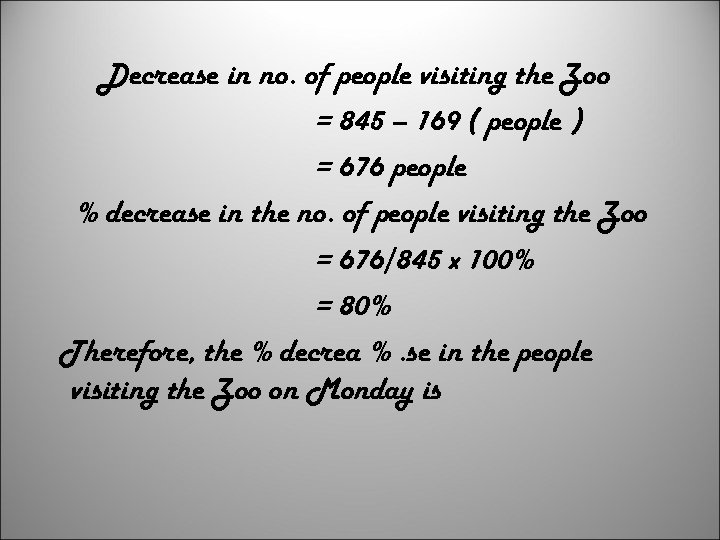Decrease in no. of people visiting the Zoo = 845 – 169 ( people ) = 676 people % decrease in the no. of people visiting the Zoo = 676/845 x 100% = 80% Therefore, the % decrea %. se in the people visiting the Zoo on Monday is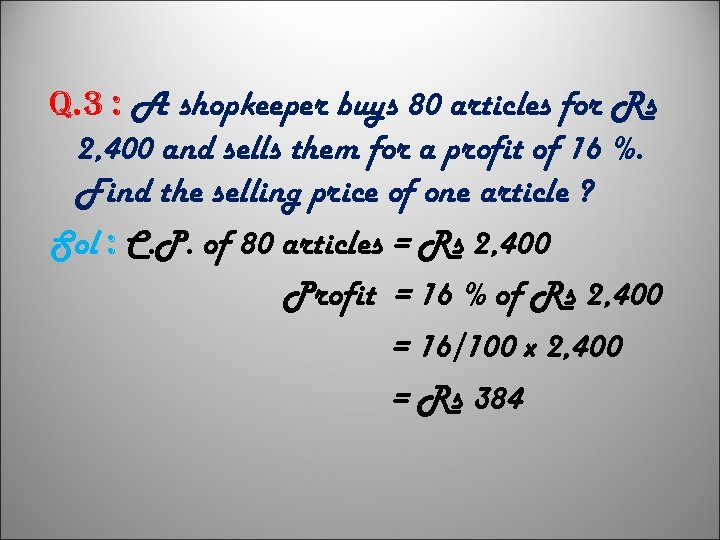q. 3 : A shopkeeper buys 80 articles for Rs 2, 400 and sells them for a profit of 16 %. Find the selling price of one article ? Sol : C. P. of 80 articles = Rs 2, 400 Profit = 16 % of Rs 2, 400 = 16/100 x 2, 400 = Rs 384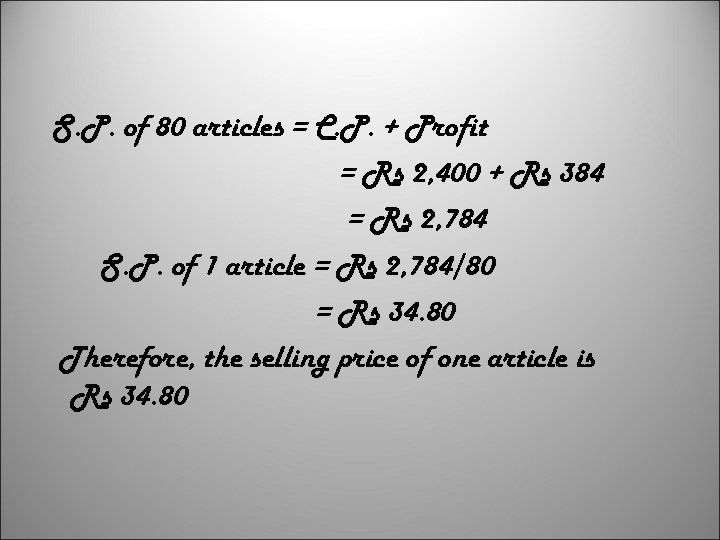S. P. of 80 articles = C. P. + Profit = Rs 2, 400 + Rs 384 = Rs 2, 784 S. P. of 1 article = Rs 2, 784/80 = Rs 34. 80 Therefore, the selling price of one article is Rs 34. 80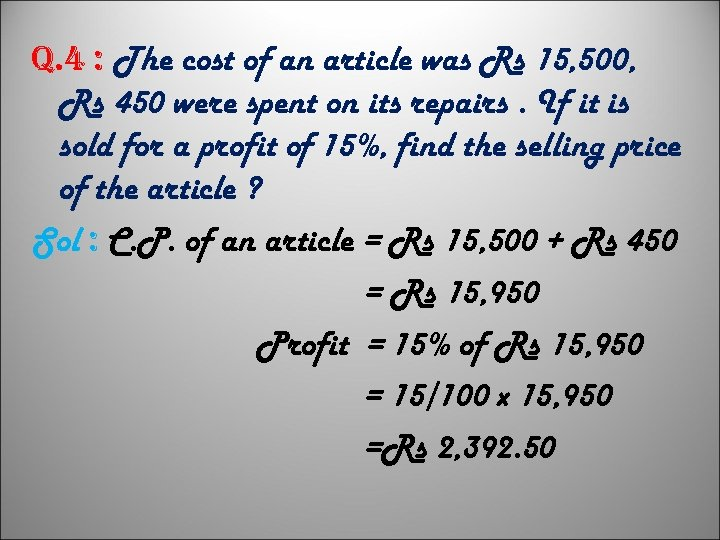q. 4 : The cost of an article was Rs 15, 500, Rs 450 were spent on its repairs. If it is sold for a profit of 15%, find the selling price of the article ? Sol : C. P. of an article = Rs 15, 500 + Rs 450 = Rs 15, 950 Profit = 15% of Rs 15, 950 = 15/100 x 15, 950 =Rs 2, 392. 50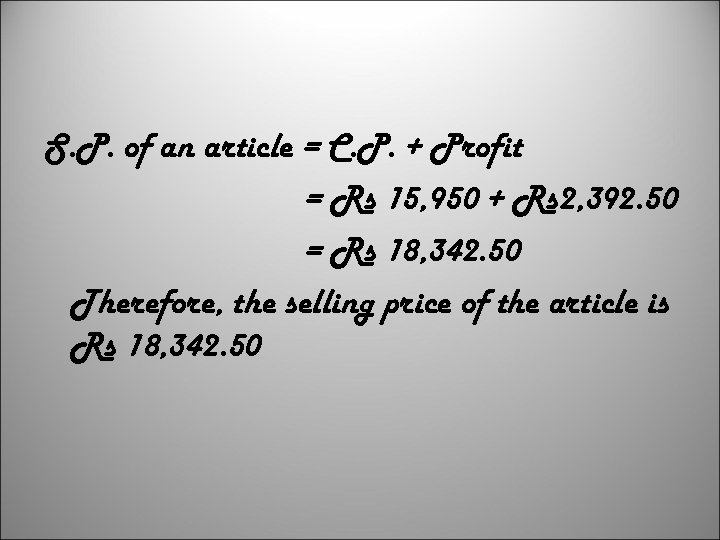S. P. of an article = C. P. + Profit = Rs 15, 950 + Rs 2, 392. 50 = Rs 18, 342. 50 Therefore, the selling price of the article is Rs 18, 342. 50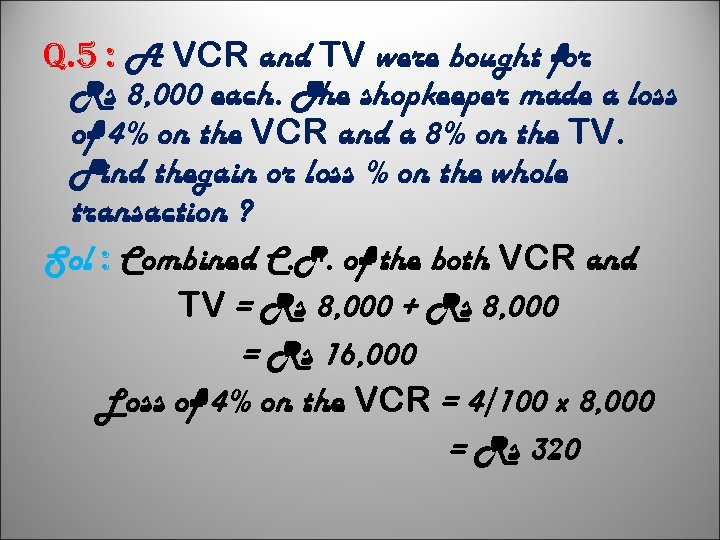q. 5 : A VCR and TV were bought for Rs 8, 000 each. The shopkeeper made a loss of 4% on the VCR and a 8% on the TV. Find thegain or loss % on the whole transaction ? Sol : Combined C. P. of the both VCR and TV = Rs 8, 000 + Rs 8, 000 = Rs 16, 000 Loss of 4% on the VCR = 4/100 x 8, 000 = Rs 320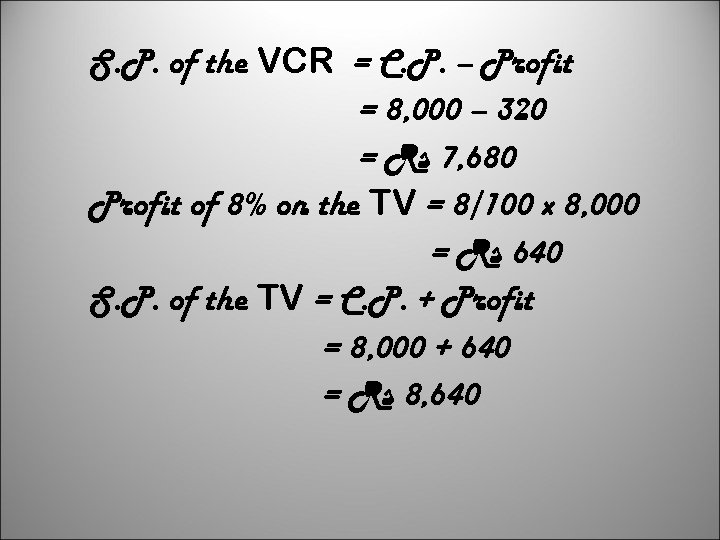S. P. of the VCR = C. P. – Profit = 8, 000 – 320 = Rs 7, 680 Profit of 8% on the TV = 8/100 x 8, 000 = Rs 640 S. P. of the TV = C. P. + Profit = 8, 000 + 640 = Rs 8, 640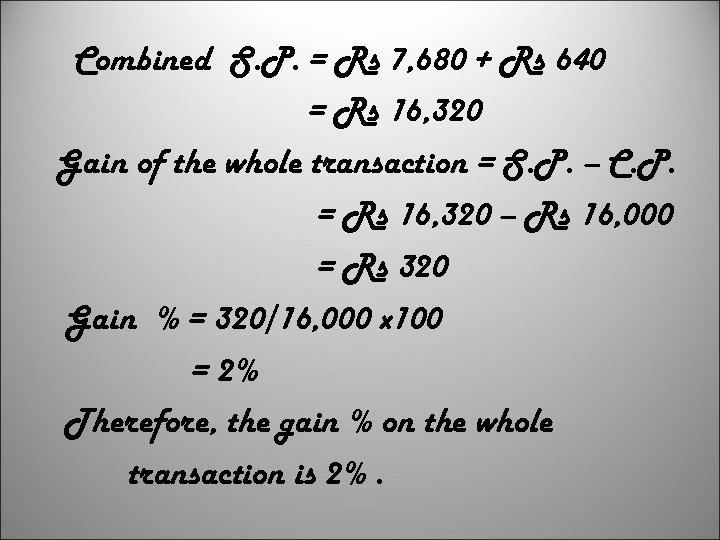Combined S. P. = Rs 7, 680 + Rs 640 = Rs 16, 320 Gain of the whole transaction = S. P. – C. P. = Rs 16, 320 – Rs 16, 000 = Rs 320 Gain % = 320/16, 000 x 100 = 2% Therefore, the gain % on the whole transaction is 2%.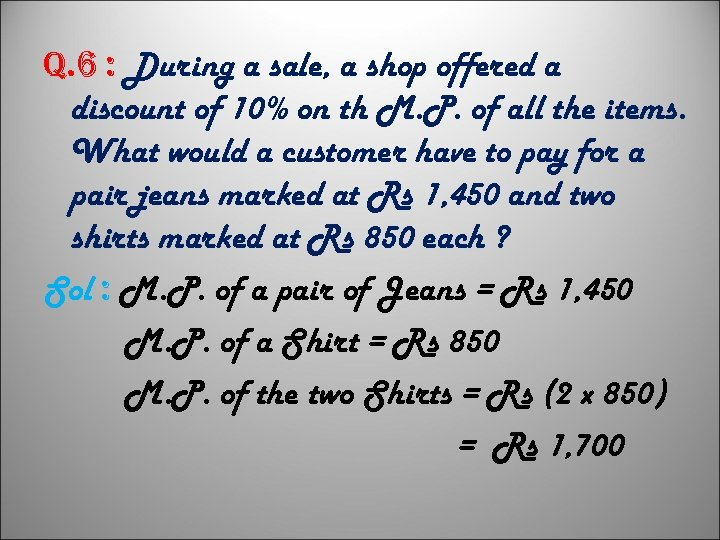q. 6 : During a sale, a shop offered a discount of 10% on th M. P. of all the items. What would a customer have to pay for a pair jeans marked at Rs 1, 450 and two shirts marked at Rs 850 each ? Sol : M. P. of a pair of Jeans = Rs 1, 450 M. P. of a Shirt = Rs 850 M. P. of the two Shirts = Rs (2 x 850) = Rs 1, 700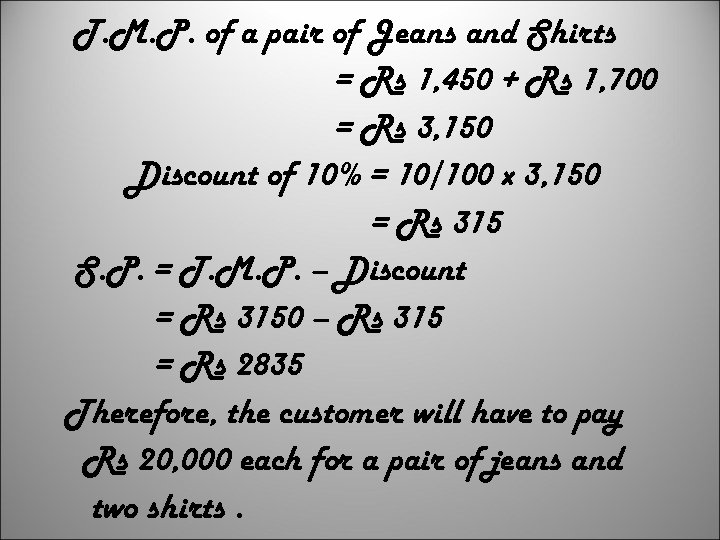T. M. P. of a pair of Jeans and Shirts = Rs 1, 450 + Rs 1, 700 = Rs 3, 150 Discount of 10% = 10/100 x 3, 150 = Rs 315 S. P. = T. M. P. – Discount = Rs 3150 – Rs 315 = Rs 2835 Therefore, the customer will have to pay Rs 20, 000 each for a pair of jeans and two shirts.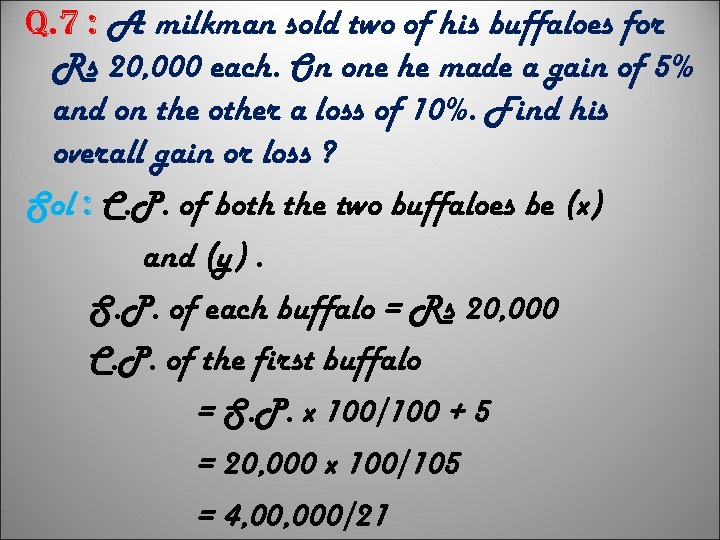q. 7 : A milkman sold two of his buffaloes for Rs 20, 000 each. On one he made a gain of 5% and on the other a loss of 10%. Find his overall gain or loss ? Sol : C. P. of both the two buffaloes be (x) and (y). S. P. of each buffalo = Rs 20, 000 C. P. of the first buffalo = S. P. x 100/100 + 5 = 20, 000 x 100/105 = 4, 000/21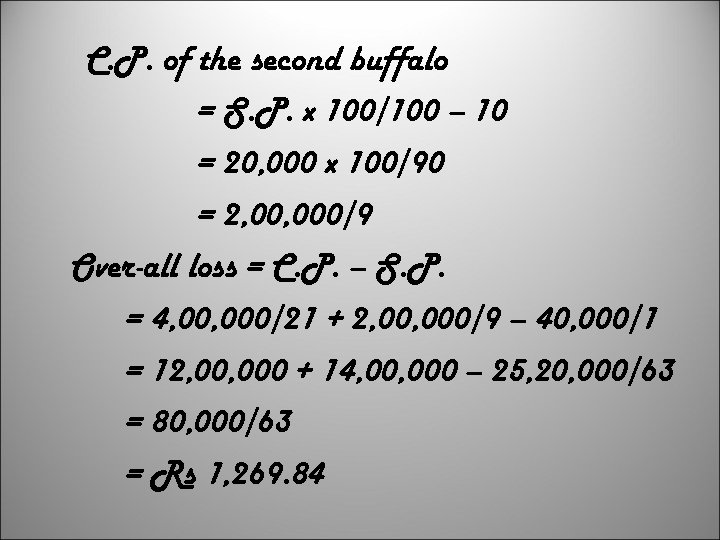C. P. of the second buffalo = S. P. x 100/100 – 10 = 20, 000 x 100/90 = 2, 000/9 Over-all loss = C. P. – S. P. = 4, 000/21 + 2, 000/9 – 40, 000/1 = 12, 000 + 14, 000 – 25, 20, 000/63 = 80, 000/63 = Rs 1, 269. 84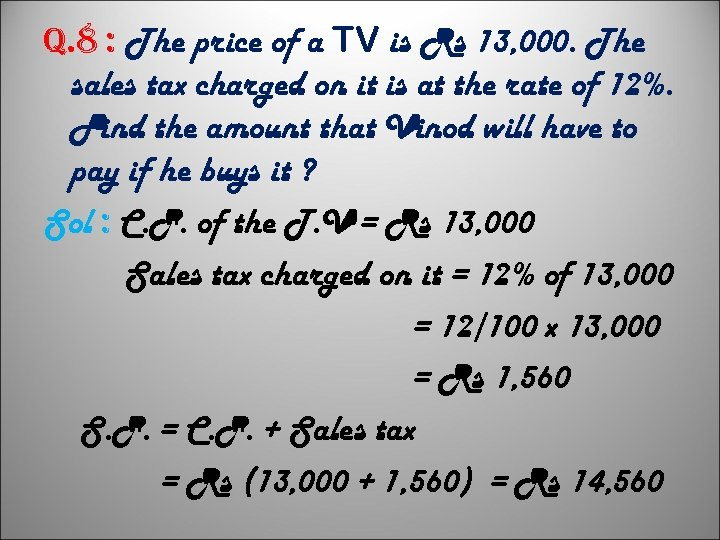q. 8 : The price of a TV is Rs 13, 000. The sales tax charged on it is at the rate of 12%. Find the amount that Vinod will have to pay if he buys it ? Sol : C. P. of the T. V = Rs 13, 000 Sales tax charged on it = 12% of 13, 000 = 12/100 x 13, 000 = Rs 1, 560 S. P. = C. P. + Sales tax = Rs (13, 000 + 1, 560) = Rs 14, 560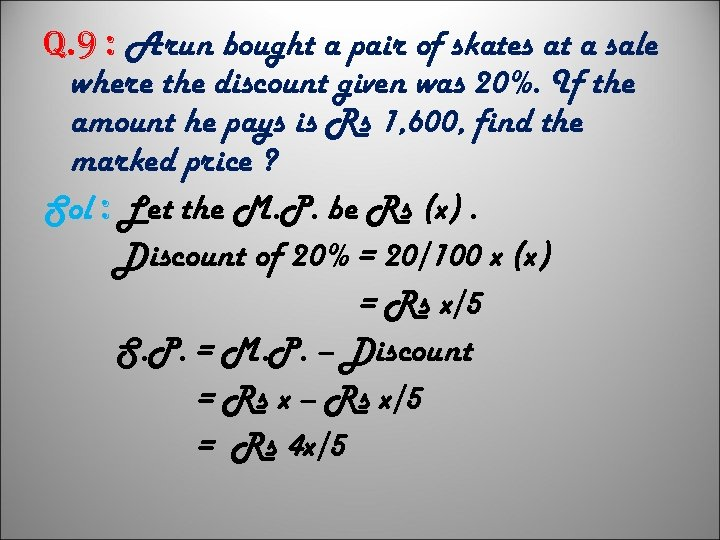q. 9 : Arun bought a pair of skates at a sale where the discount given was 20%. If the amount he pays is Rs 1, 600, find the marked price ? Sol : Let the M. P. be Rs (x). Discount of 20% = 20/100 x (x) = Rs x/5 S. P. = M. P. – Discount = Rs x – Rs x/5 = Rs 4 x/5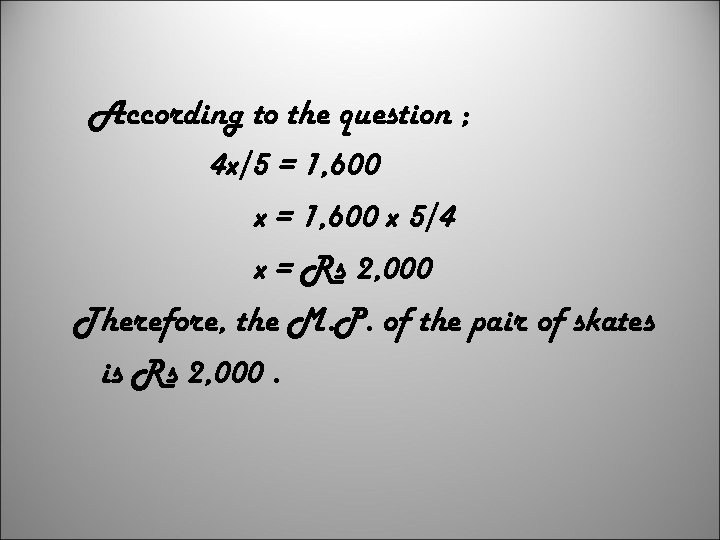According to the question ; 4 x/5 = 1, 600 x 5/4 x = Rs 2, 000 Therefore, the M. P. of the pair of skates is Rs 2, 000.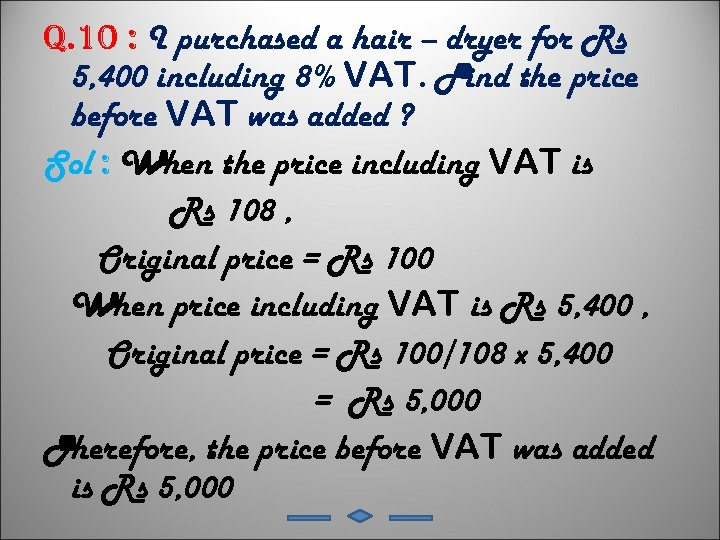q. 10 : I purchased a hair – dryer for Rs 5, 400 including 8% VAT. Find the price before VAT was added ? Sol : When the price including VAT is Rs 108 , Original price = Rs 100 When price including VAT is Rs 5, 400 , Original price = Rs 100/108 x 5, 400 = Rs 5, 000 Therefore, the price before VAT was added is Rs 5, 000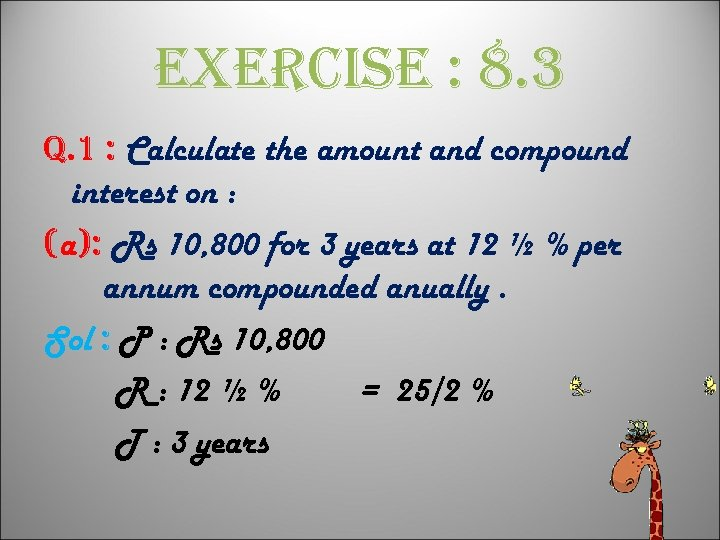exercise : 8. 3 q. 1 : Calculate the amount and compound interest on : (a): Rs 10, 800 for 3 years at 12 ½ % per annum compounded anually. Sol : P : Rs 10, 800 R : 12 ½ % = 25/2 % T : 3 years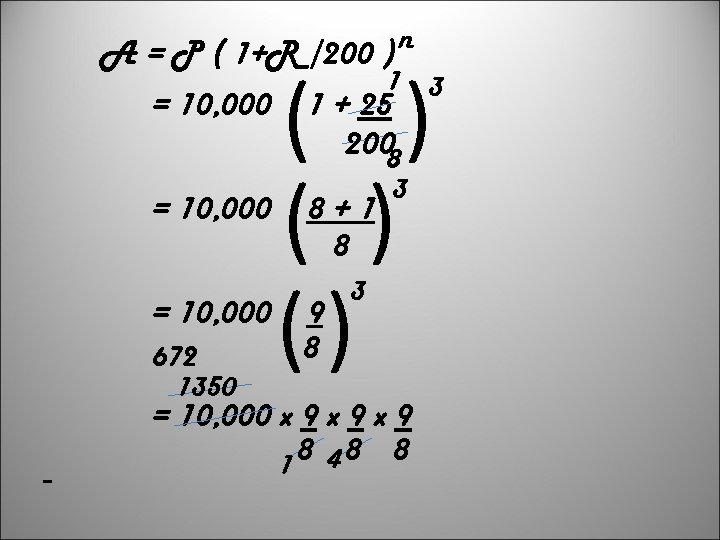A = P ( 1+R /200 ) n 1 3 = 10, 000 1 + 25 200 8 = 10, 000 672 1350 ( ) () 8+1 8 9 8 3 3 = 10, 000 x 9 x 9 8 48 8 1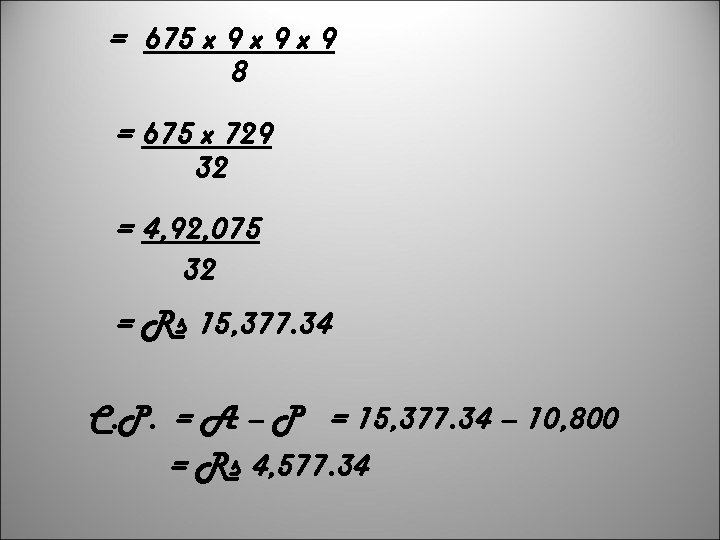= 675 x 9 x 9 8 = 675 x 729 32 = 4, 92, 075 32 = Rs 15, 377. 34 C. P. = A – P = 15, 377. 34 – 10, 800 = Rs 4, 577. 34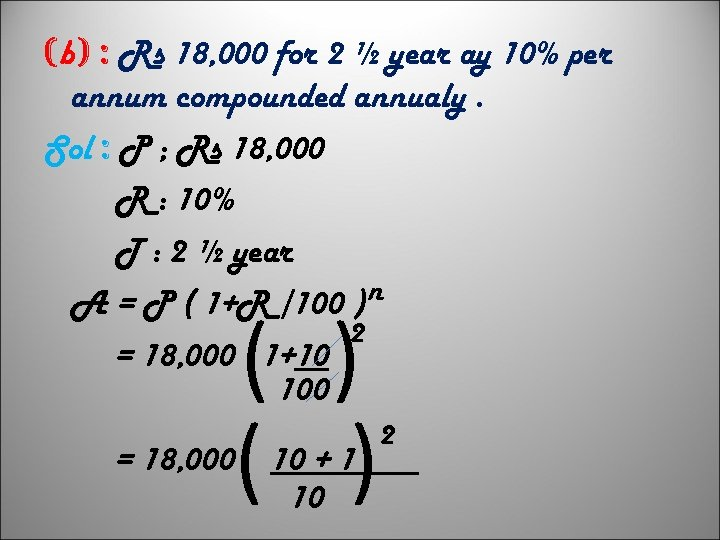(b) : Rs 18, 000 for 2 ½ year ay 10% per annum compounded annualy. Sol : P ; Rs 18, 000 R : 10% T : 2 ½ year A = P ( 1+R /100 ) n 2 = 18, 000 1+10 100 = 18, 000 ( ) 10 + 1 10 2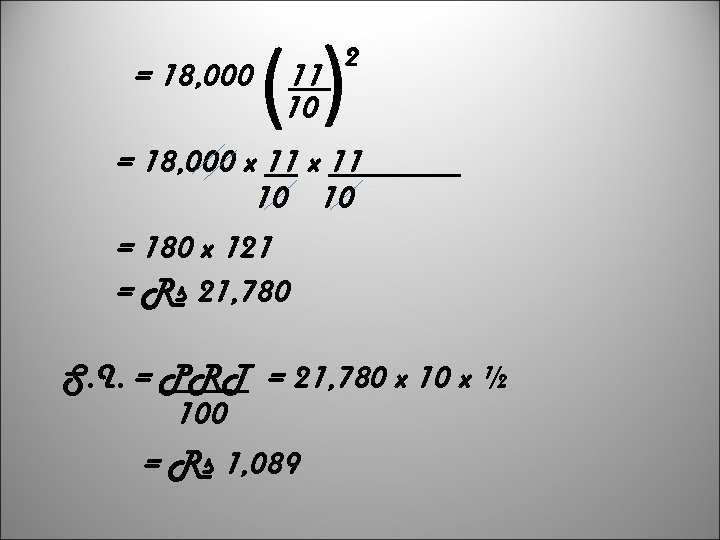= 18, 000 () 11 10 2 = 18, 000 x 11 10 10 = 180 x 121 = Rs 21, 780 S. I. = PRT = 21, 780 x 10 x ½ 100 = Rs 1, 089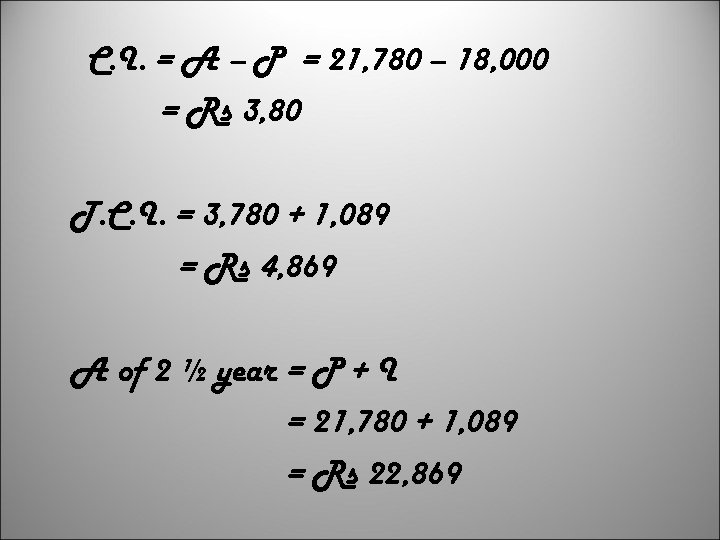C. I. = A – P = 21, 780 – 18, 000 = Rs 3, 80 T. C. I. = 3, 780 + 1, 089 = Rs 4, 869 A of 2 ½ year = P + I = 21, 780 + 1, 089 = Rs 22, 869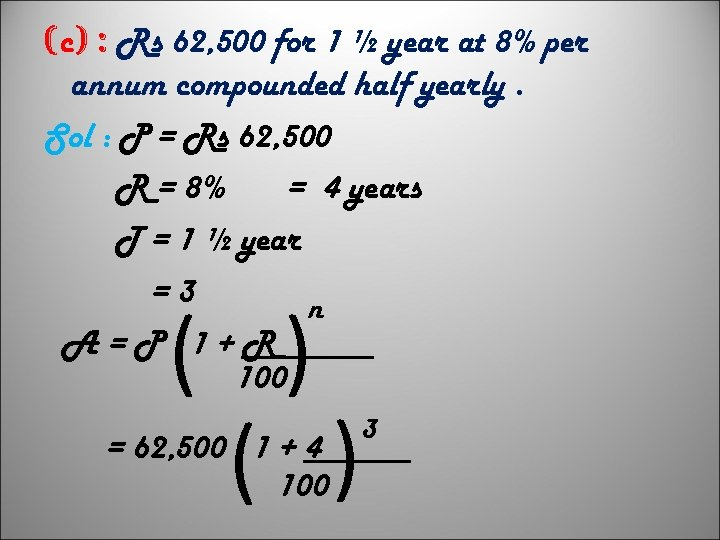(c) : Rs 62, 500 for 1 ½ year at 8% per annum compounded half yearly. Sol : P = Rs 62, 500 R = 8% = 4 years T = 1 ½ year =3 n A=P 1+R 100 ( ) = 62, 500 1 + 4 100 3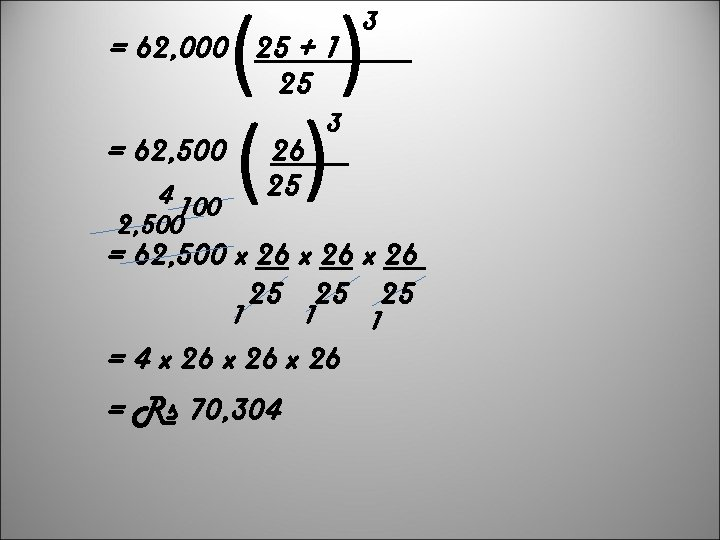( ) () = 62, 000 25 + 1 25 = 62, 500 4 100 2, 500 26 25 3 3 = 62, 500 x 26 25 25 25 1 1 = 4 x 26 = Rs 70, 304 1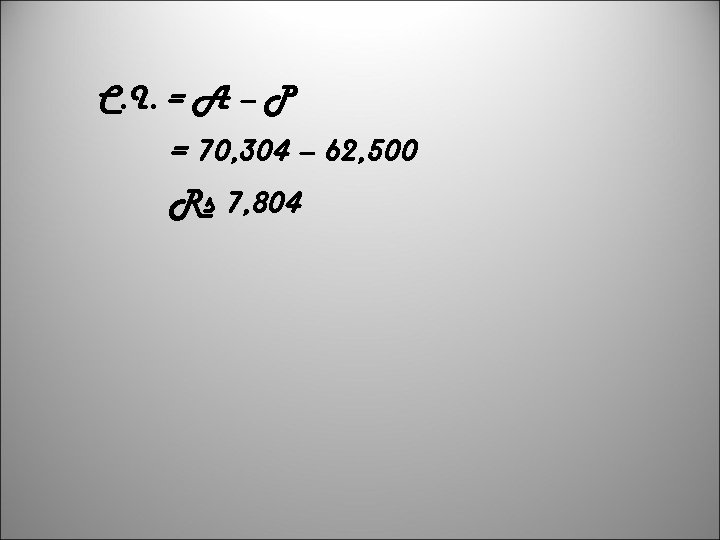C. I. = A – P = 70, 304 – 62, 500 Rs 7, 804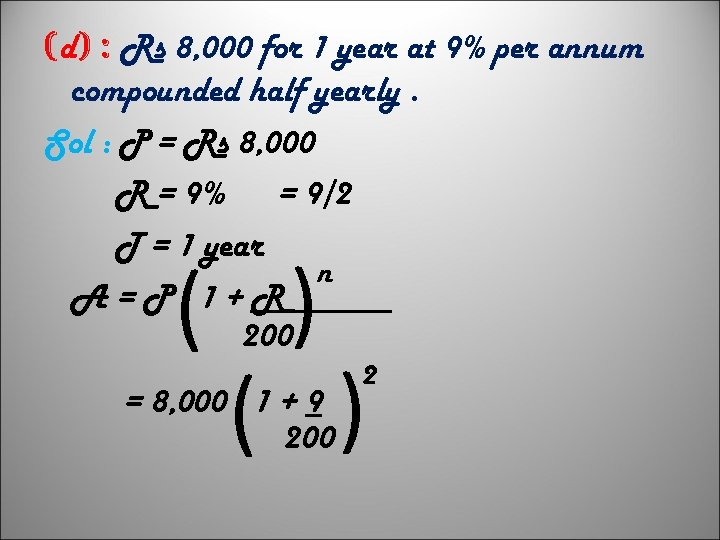(d) : Rs 8, 000 for 1 year at 9% per annum compounded half yearly. Sol : P = Rs 8, 000 R = 9% = 9/2 T = 1 year n A=P 1+R 200 ( ) = 8, 000 1 + 9 200 2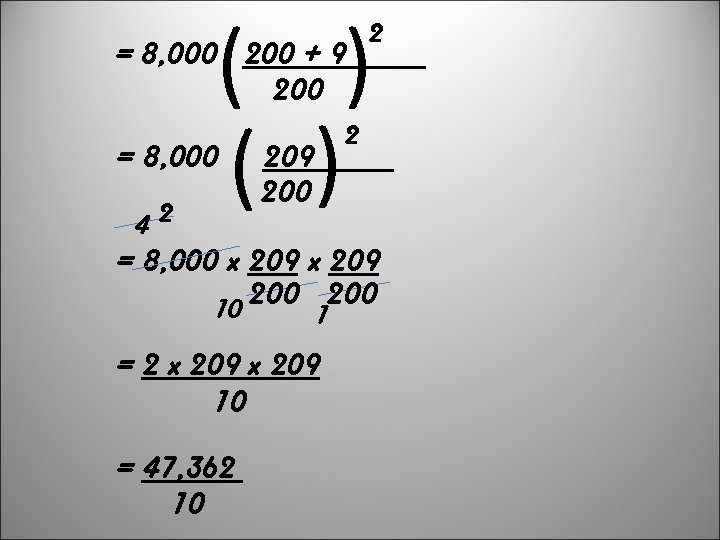( ) = 8, 000 200 + 9 200 = 8, 000 42 2 2 209 200 = 8, 000 x 209 200 10 1 = 2 x 209 10 = 47, 362 10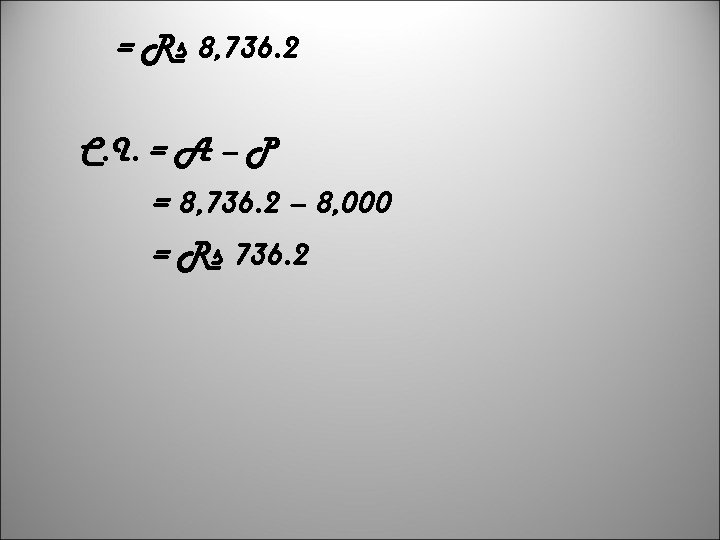= Rs 8, 736. 2 C. I. = A – P = 8, 736. 2 – 8, 000 = Rs 736. 2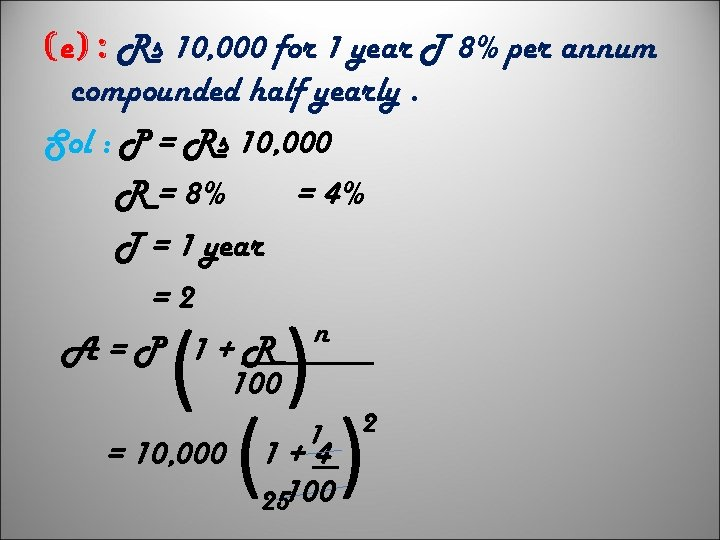(e) : Rs 10, 000 for 1 year T 8% per annum compounded half yearly. Sol : P = Rs 10, 000 R = 8% = 4% T = 1 year =2 n A=P 1+R 100 ( ) = 10, 000 1 1+4 25100 2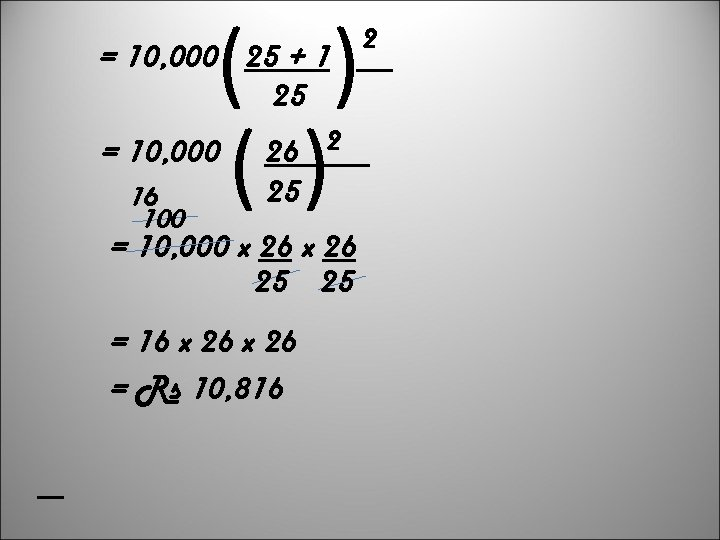( ) = 10, 000 25 + 1 25 = 10, 000 16 100 26 2 25 = 10, 000 x 26 25 25 = 16 x 26 = Rs 10, 816 2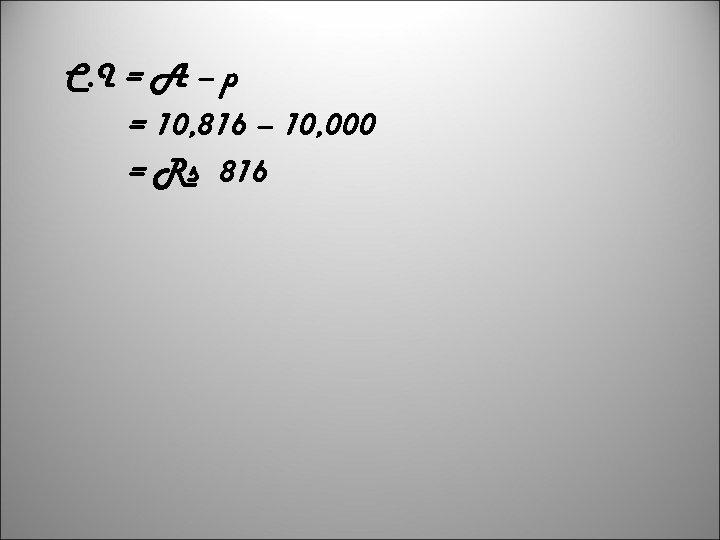C. I = A – p = 10, 816 – 10, 000 = Rs 816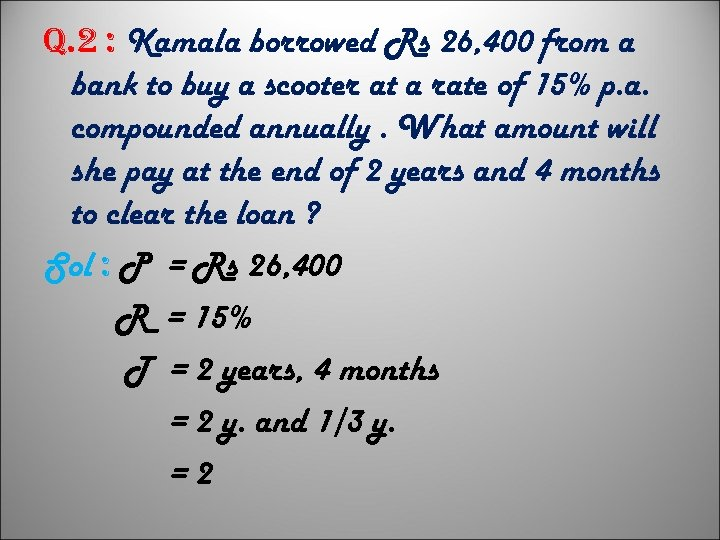q. 2 : Kamala borrowed Rs 26, 400 from a bank to buy a scooter at a rate of 15% p. a. compounded annually. What amount will she pay at the end of 2 years and 4 months to clear the loan ? Sol : P = Rs 26, 400 R = 15% T = 2 years, 4 months = 2 y. and 1/3 y. =2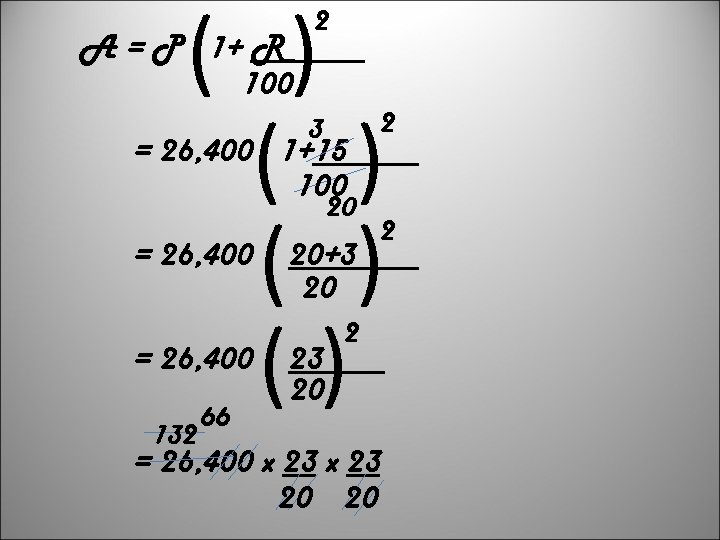( ) ( ) () A = P 1+ R 100 2 3 = 26, 400 1+15 100 20 = 26, 400 132 66 20+3 20 2 2 2 = 26, 400 x 23 20 20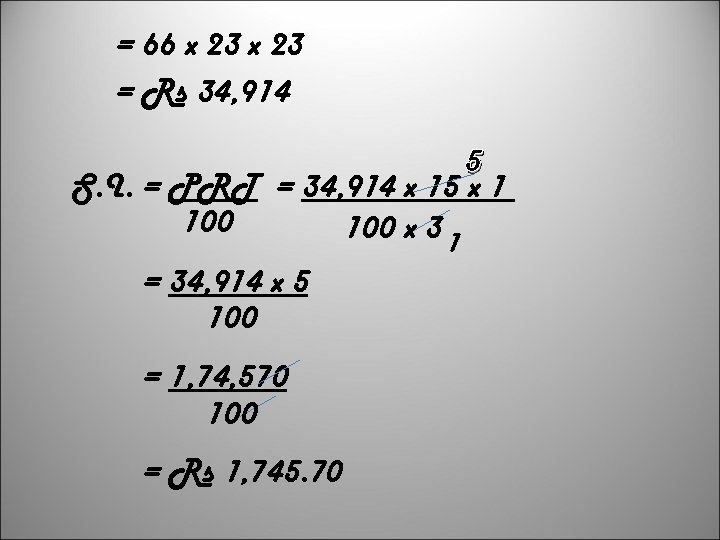= 66 x 23 = Rs 34, 914 5 S. I. = PRT = 34, 914 x 15 x 1 100 x 3 1 = 34, 914 x 5 100 = 1, 74, 570 100 = Rs 1, 745. 70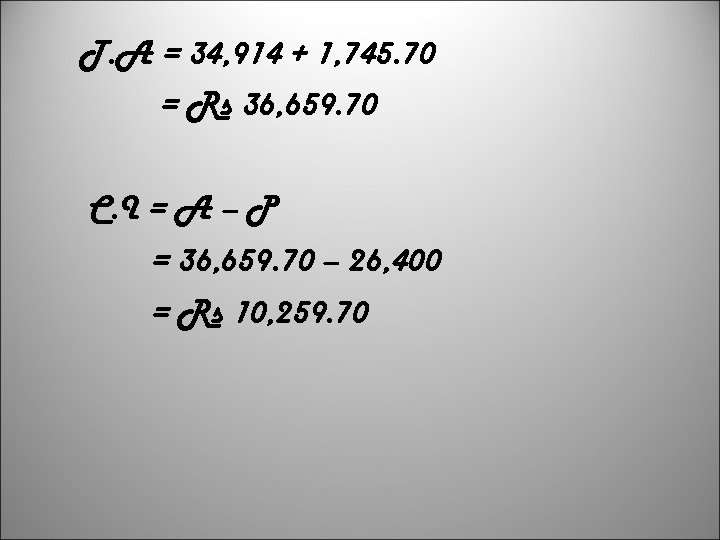T. A = 34, 914 + 1, 745. 70 = Rs 36, 659. 70 C. I = A – P = 36, 659. 70 – 26, 400 = Rs 10, 259. 70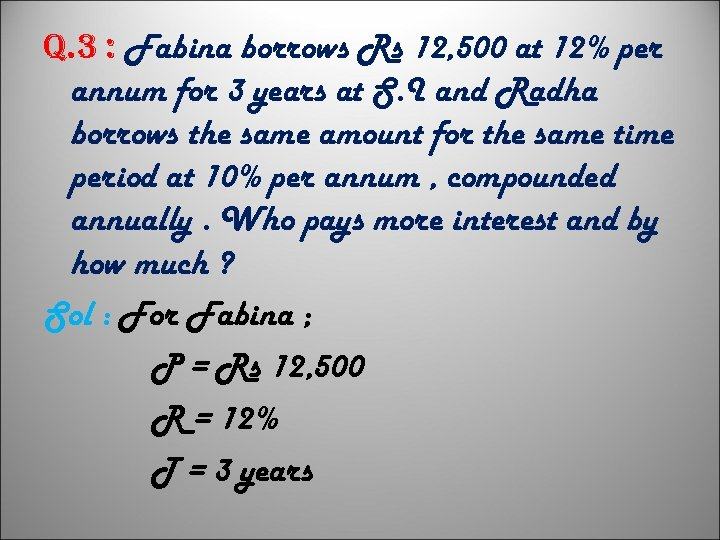q. 3 : Fabina borrows Rs 12, 500 at 12% per annum for 3 years at S. I and Radha borrows the same amount for the same time period at 10% per annum , compounded annually. Who pays more interest and by how much ? Sol : For Fabina ; P = Rs 12, 500 R = 12% T = 3 years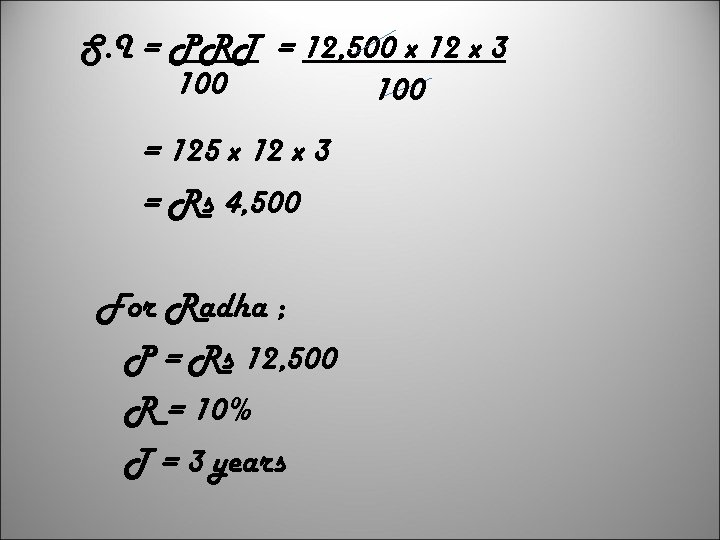S. I = PRT = 12, 500 x 12 x 3 100 = 125 x 12 x 3 = Rs 4, 500 For Radha ; P = Rs 12, 500 R = 10% T = 3 years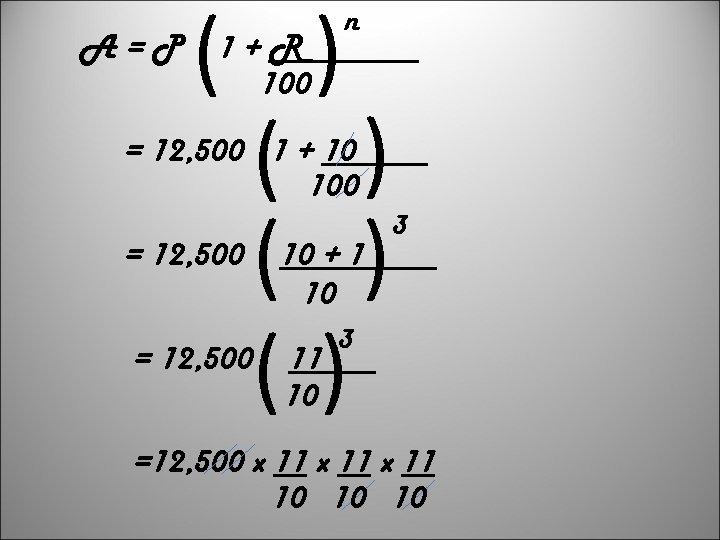( ) ( ) () A=P 1+R 100 n = 12, 500 1 + 10 100 = 12, 500 10 + 1 10 11 10 3 3 =12, 500 x 11 10 10 10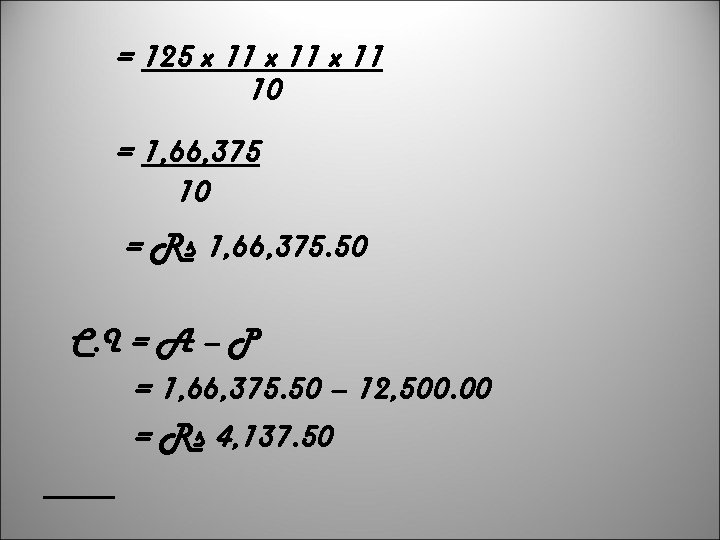= 125 x 11 10 = 1, 66, 375 10 = Rs 1, 66, 375. 50 C. I = A – P = 1, 66, 375. 50 – 12, 500. 00 = Rs 4, 137. 50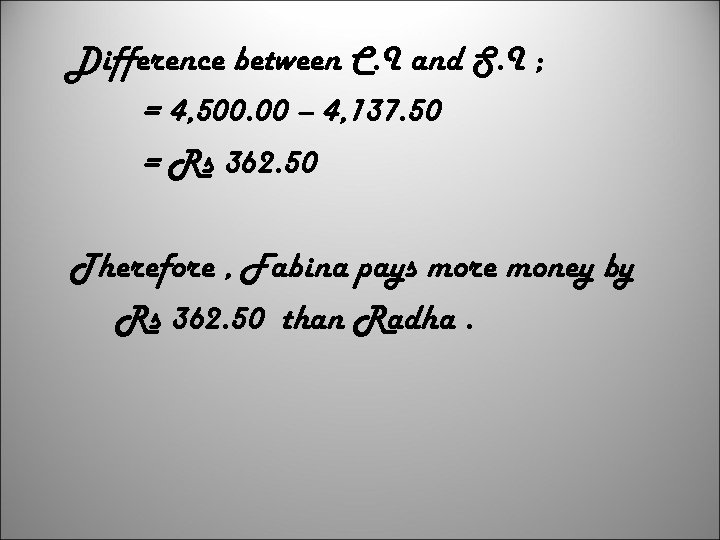Difference between C. I and S. I ; = 4, 500. 00 – 4, 137. 50 = Rs 362. 50 Therefore , Fabina pays more money by Rs 362. 50 than Radha.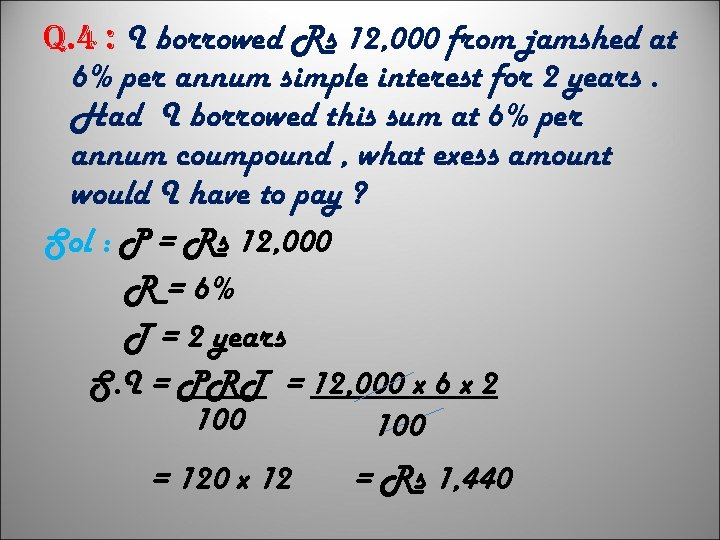q. 4 : I borrowed Rs 12, 000 from jamshed at 6% per annum simple interest for 2 years. Had I borrowed this sum at 6% per annum coumpound , what exess amount would I have to pay ? Sol : P = Rs 12, 000 R = 6% T = 2 years S. I = PRT = 12, 000 x 6 x 2 100 = 120 x 12 = Rs 1, 440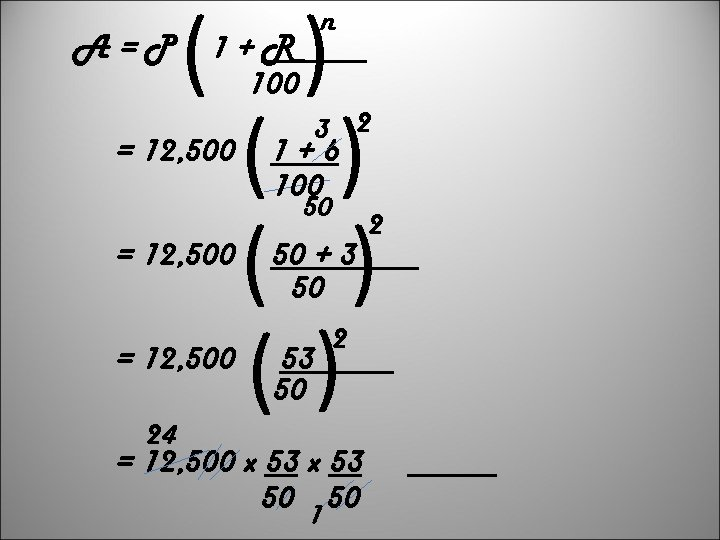A=P ( ) ( ) () n 1+R 100 = 12, 500 3 2 1+6 100 50 = 12, 500 24 50 + 3 50 53 50 2 = 12, 500 x 53 50 1 50 2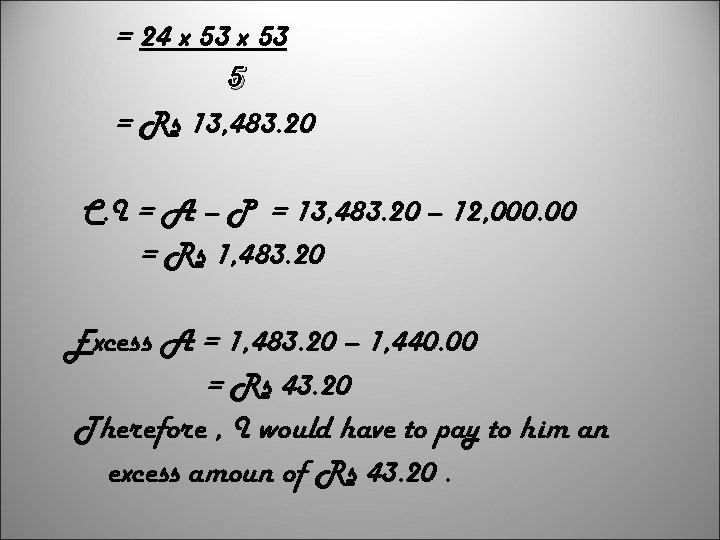= 24 x 53 5 = Rs 13, 483. 20 C. I = A – P = 13, 483. 20 – 12, 000. 00 = Rs 1, 483. 20 Excess A = 1, 483. 20 – 1, 440. 00 = Rs 43. 20 Therefore , I would have to pay to him an excess amoun of Rs 43. 20.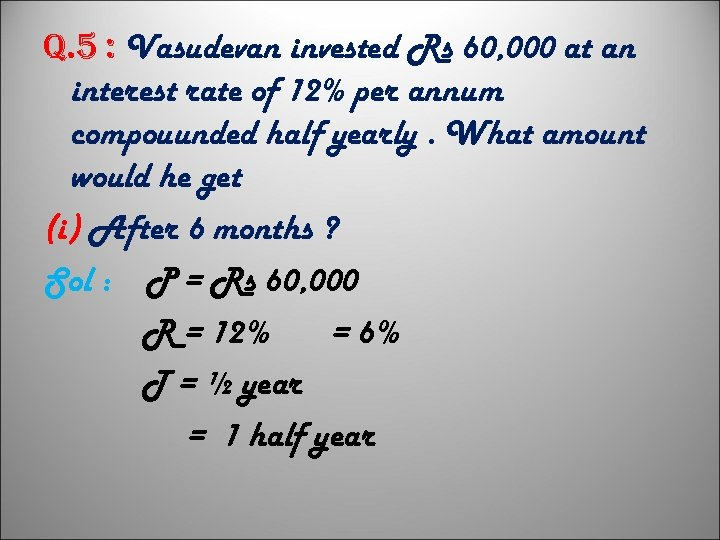q. 5 : Vasudevan invested Rs 60, 000 at an interest rate of 12% per annum compouunded half yearly. What amount would he get (i) After 6 months ? Sol : P = Rs 60, 000 R = 12% = 6% T = ½ year = 1 half year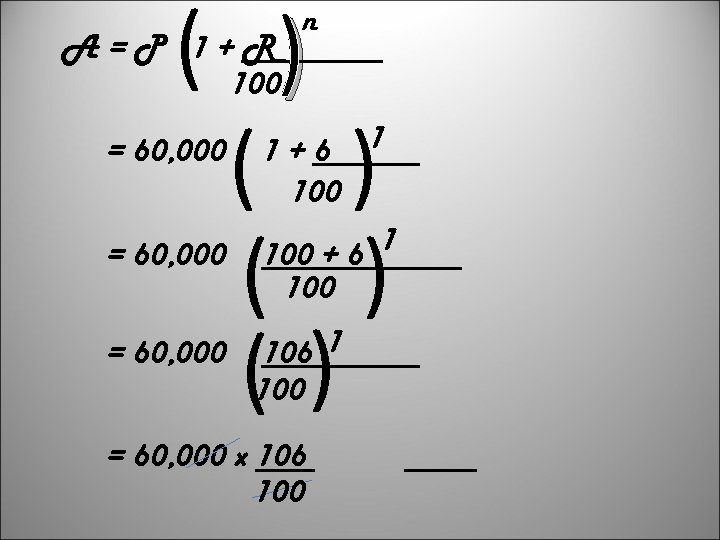( ( ( A=P 1+R 100 = 60, 000 n ( ) () 1+6 100 1 1 = 60, 000 100 + 6 100 = 60, 000 106 1 100 = 60, 000 x 106 100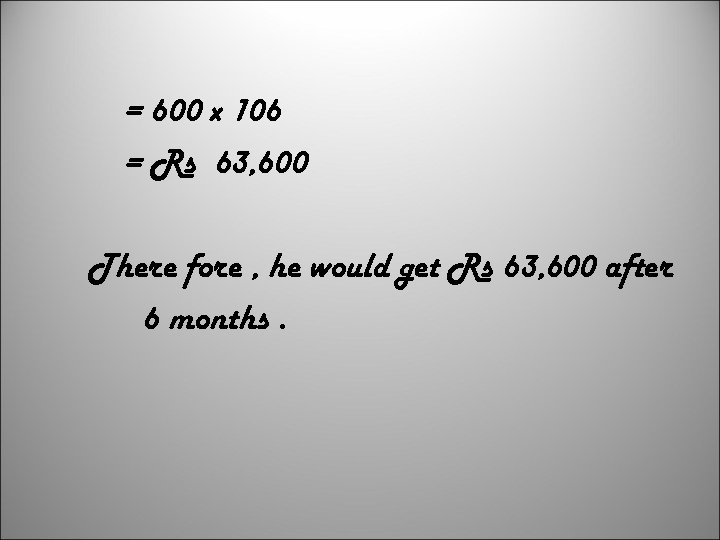= 600 x 106 = Rs 63, 600 There fore , he would get Rs 63, 600 after 6 months.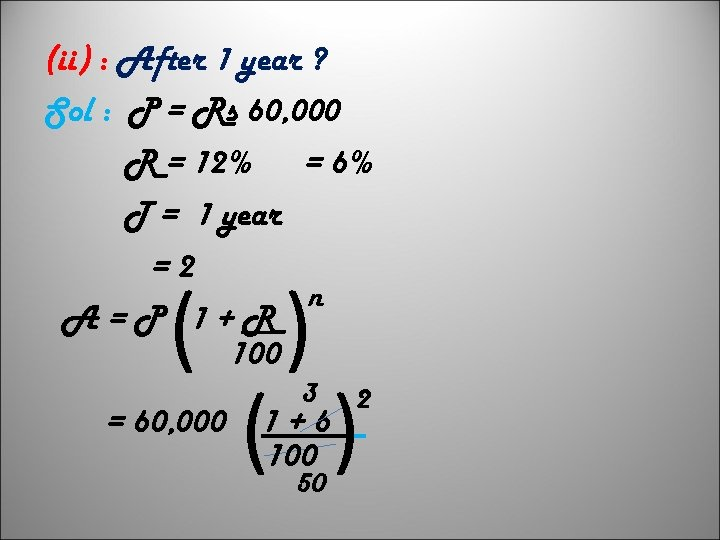(ii) : After 1 year ? Sol : P = Rs 60, 000 R = 12% = 6% T = 1 year =2 n A=P 1+R 100 ( ) = 60, 000 3 1+6 100 50 2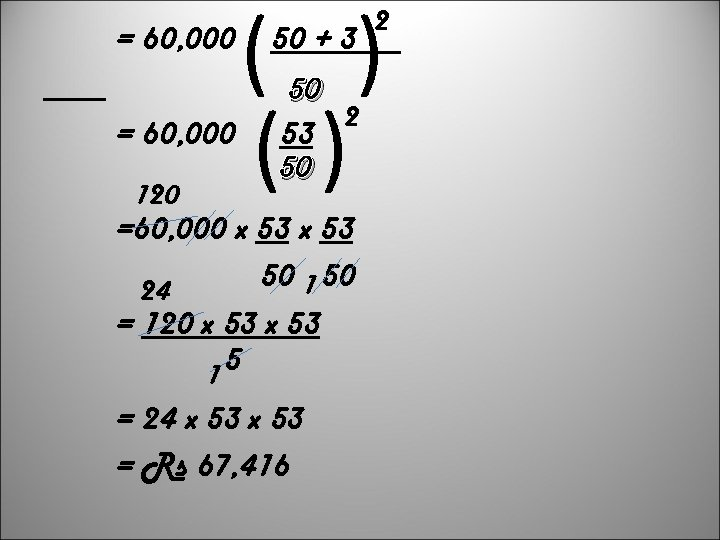= 60, 000 120 ( ) () 50 + 3 50 2 53 50 =60, 000 x 53 50 1 50 24 = 120 x 53 5 1 = 24 x 53 = Rs 67, 416 2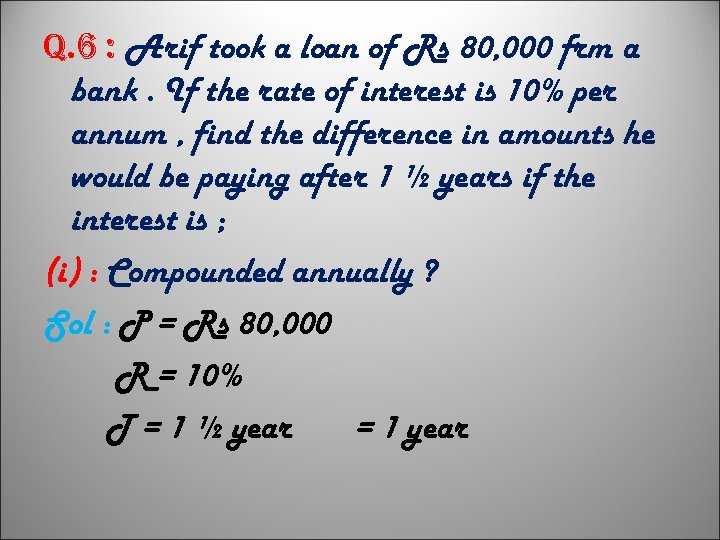q. 6 : Arif took a loan of Rs 80, 000 frm a bank. If the rate of interest is 10% per annum , find the difference in amounts he would be paying after 1 ½ years if the interest is ; (i) : Compounded annually ? Sol : P = Rs 80, 000 R = 10% T = 1 ½ year = 1 year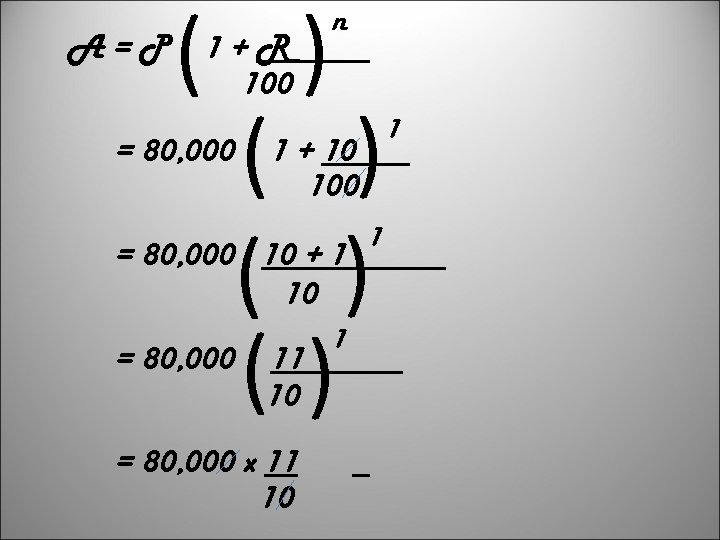( ( ( = 80, 000 ( ( A=P 1+R 100 n 1 + 10 100 = 80, 000 10 + 1 10 11 10 = 80, 000 x 11 10 ( = 80, 000 1 1 1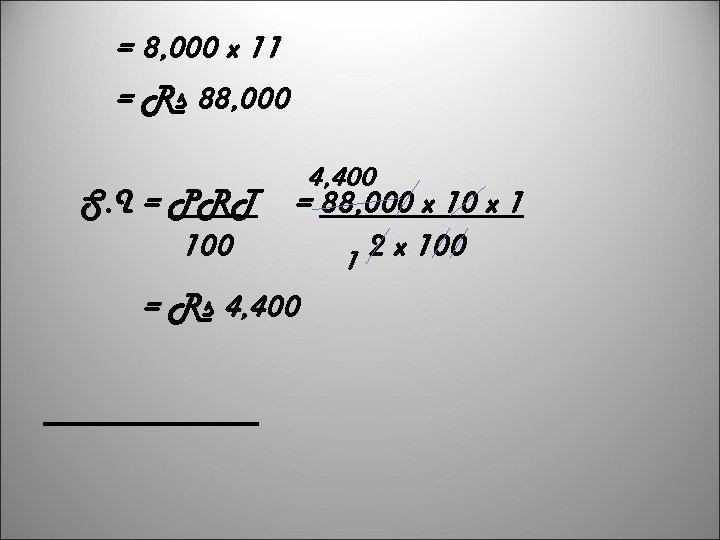= 8, 000 x 11 = Rs 88, 000 S. I = PRT 100 4, 400 = 88, 000 x 1 2 x 100 1 = Rs 4, 400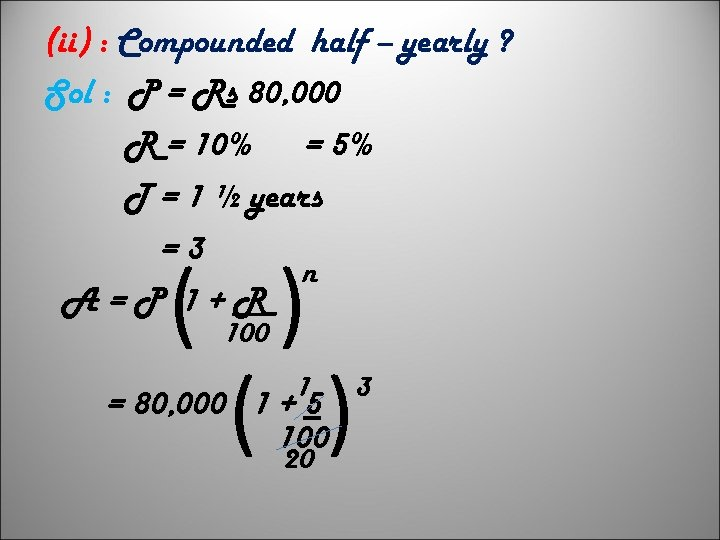(ii) : Compounded half – yearly ? Sol : P = Rs 80, 000 R = 10% = 5% T = 1 ½ years =3 n A=P 1+R ( ) 100 1 = 80, 000 1 + 5 100 20 3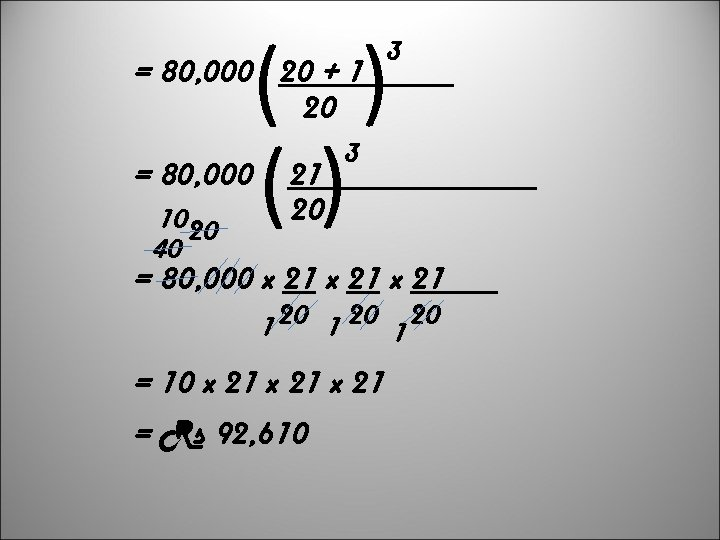( ( ( = 80, 000 20 + 1 20 1020 40 ( = 80, 000 3 21 20 3 = 80, 000 x 21 1 20 = 10 x 21 = Rs 92, 610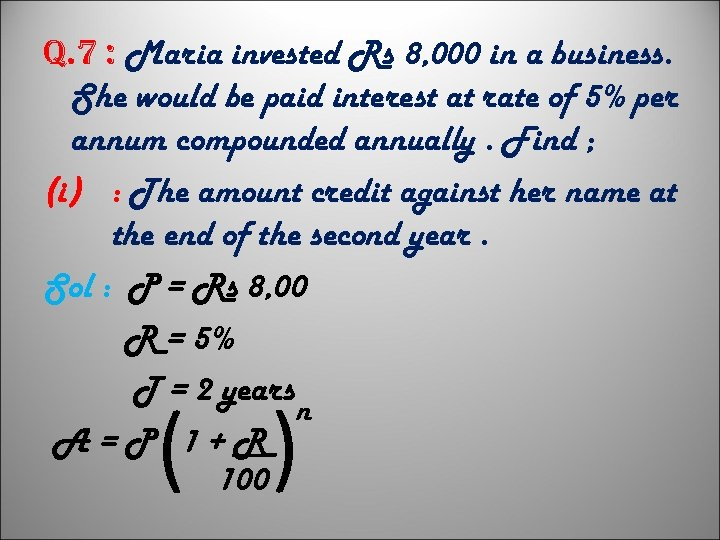q. 7 : Maria invested Rs 8, 000 in a business. She would be paid interest at rate of 5% per annum compounded annually. Find ; (i) : The amount credit against her name at the end of the second year. Sol : P = Rs 8, 00 R = 5% T = 2 years n A=P 1+R 100 ( (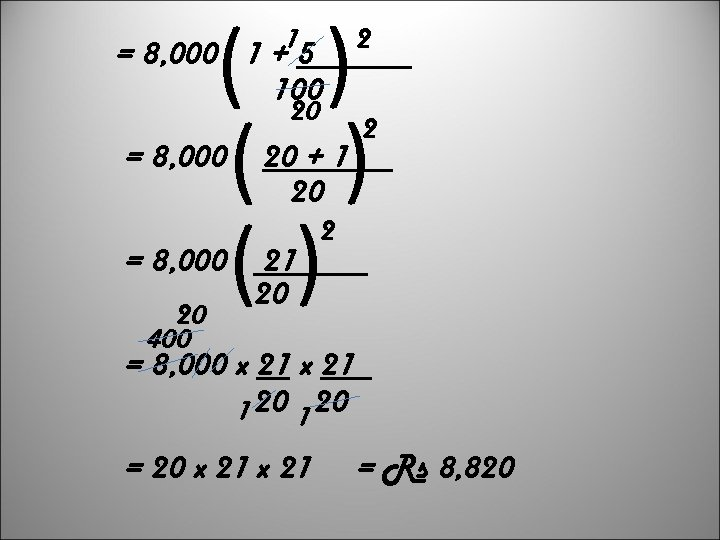( ( ( = 8, 000 1 +15 2 100 = 8, 000 20 400 20 + 1 20 2 2 ( = 8, 000 20 = 8, 000 x 21 1 20 = 20 x 21 = Rs 8, 820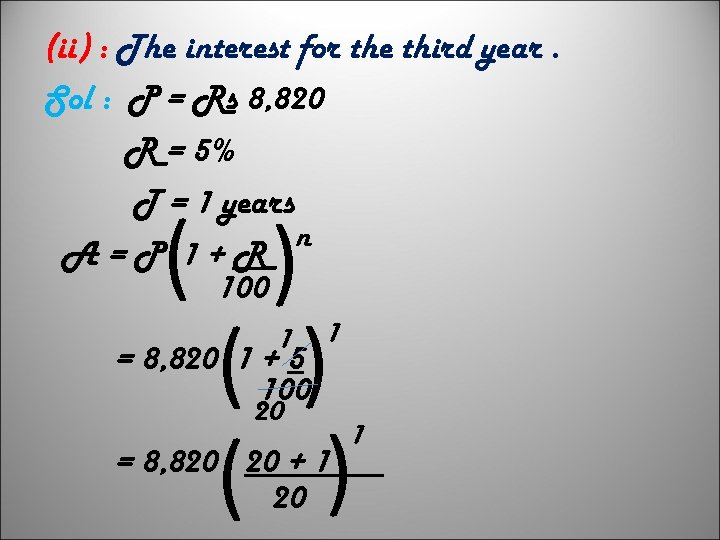(ii) : The interest for the third year. Sol : P = Rs 8, 820 R = 5% T = 1 years n A=P 1+R 100 1 = 8, 820 1 + 5 100 20 1 ( ( ( = 8, 820 20 + 1 20 1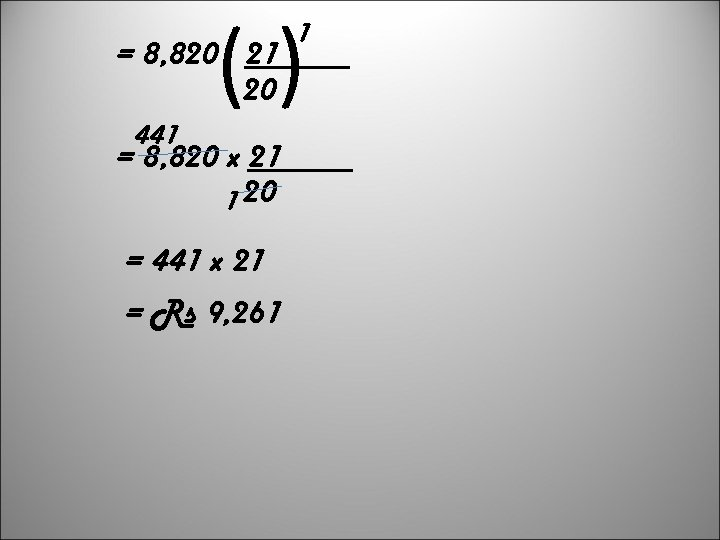( ( = 8, 820 21 20 441 1 = 8, 820 x 21 1 20 = 441 x 21 = Rs 9, 261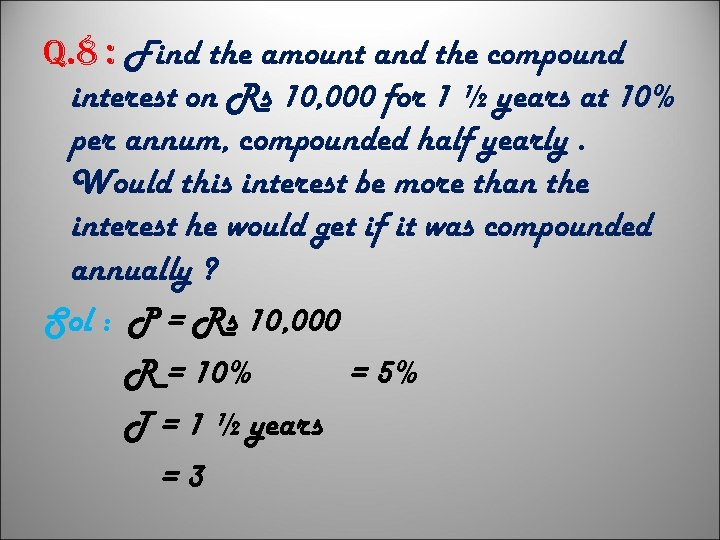q. 8 : Find the amount and the compound interest on Rs 10, 000 for 1 ½ years at 10% per annum, compounded half yearly. Would this interest be more than the interest he would get if it was compounded annually ? Sol : P = Rs 10, 000 R = 10% = 5% T = 1 ½ years =3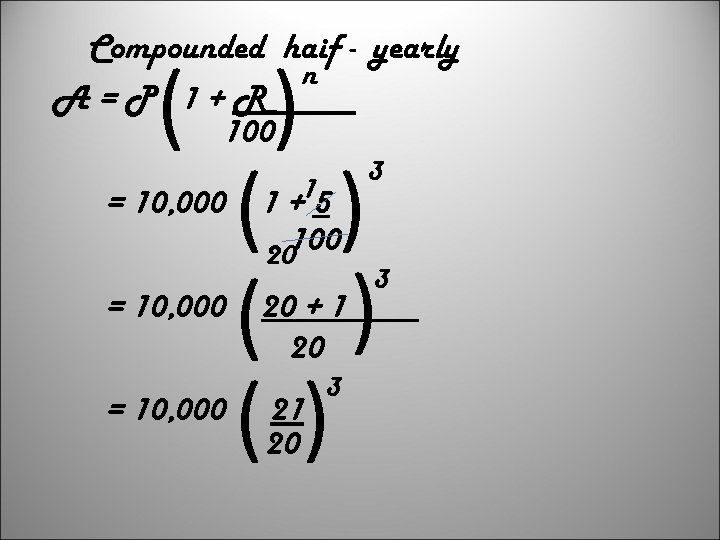Compounded haif - yearly n A=P 1+R 100 ( ) ( ) () = 10, 000 15 1+ 100 20 20 + 1 20 21 20 3 3 3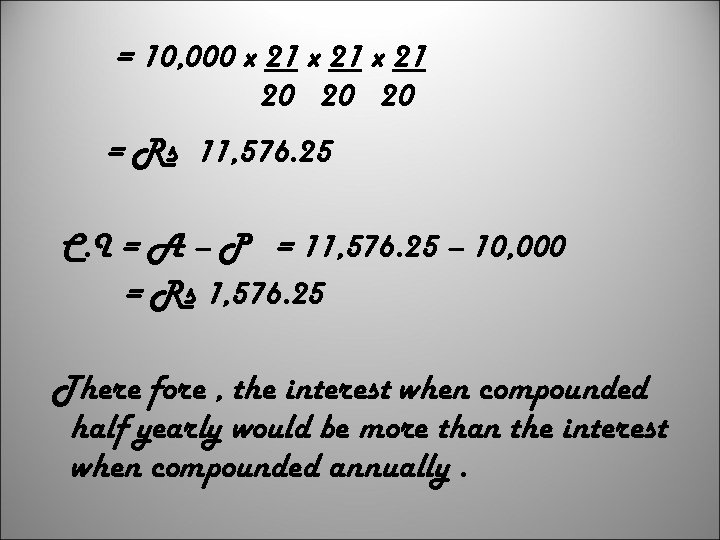= 10, 000 x 21 20 20 20 = Rs 11, 576. 25 C. I = A – P = 11, 576. 25 – 10, 000 = Rs 1, 576. 25 There fore , the interest when compounded half yearly would be more than the interest when compounded annually.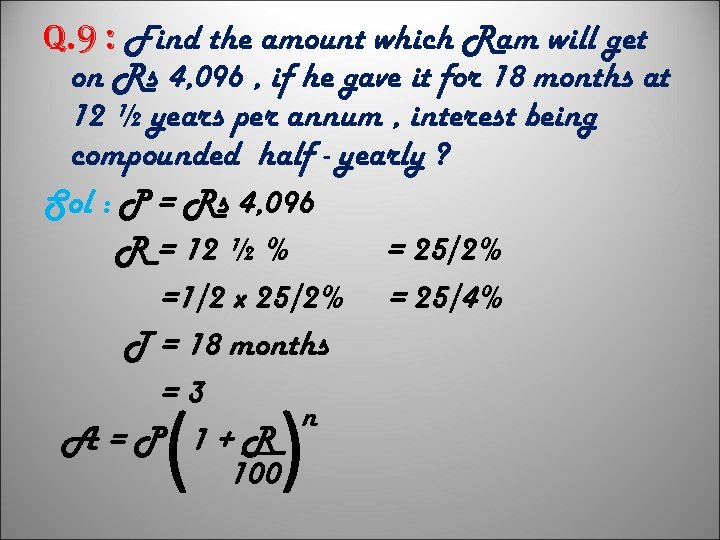q. 9 : Find the amount which Ram will get on Rs 4, 096 , if he gave it for 18 months at 12 ½ years per annum , interest being compounded half - yearly ? Sol : P = Rs 4, 096 R = 12 ½ % = 25/2% =1/2 x 25/2% = 25/4% T = 18 months =3 n A=P 1+R 100 ( )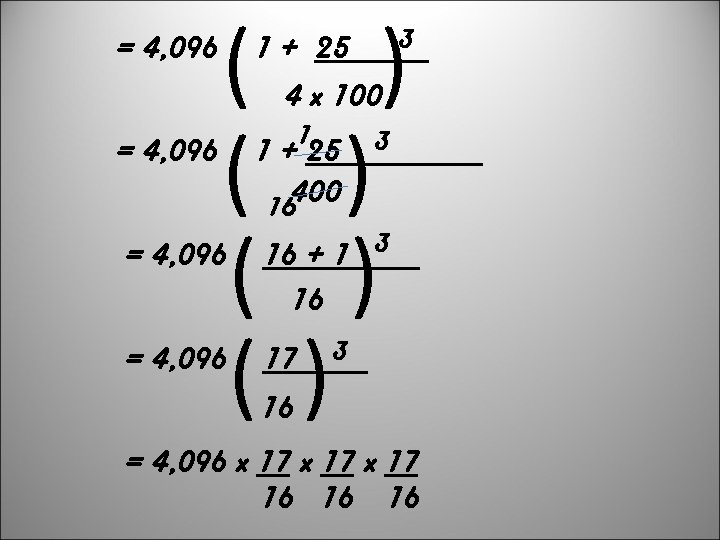= 4, 096 ( ) ( ) 1 + 25 3 4 x 100 1 1 + 25 3 400 16 = 4, 096 16 + 1 3 16 = 4, 096 17 3 16 = 4, 096 x 17 16 16 16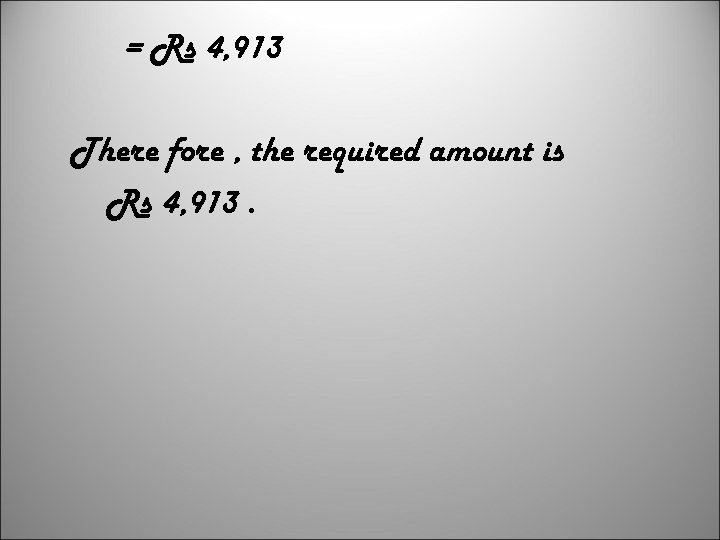= Rs 4, 913 There fore , the required amount is Rs 4, 913.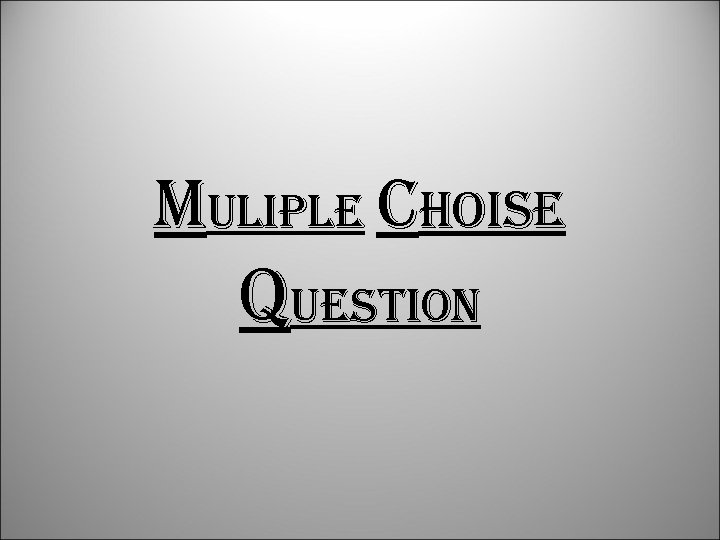Muliple choise question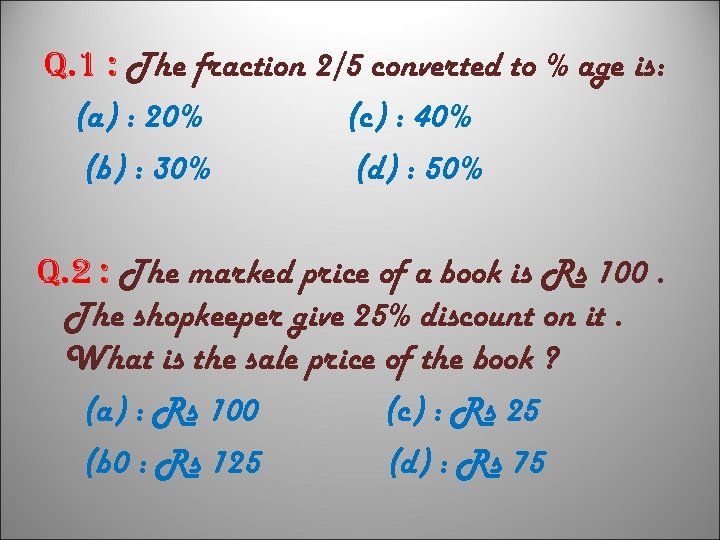q. 1 : The fraction 2/5 converted to % age is: (a) : 20% (c) : 40% (b) : 30% (d) : 50% q. 2 : The marked price of a book is Rs 100. The shopkeeper give 25% discount on it. What is the sale price of the book ? (a) : Rs 100 (c) : Rs 25 (b 0 : Rs 125 (d) : Rs 75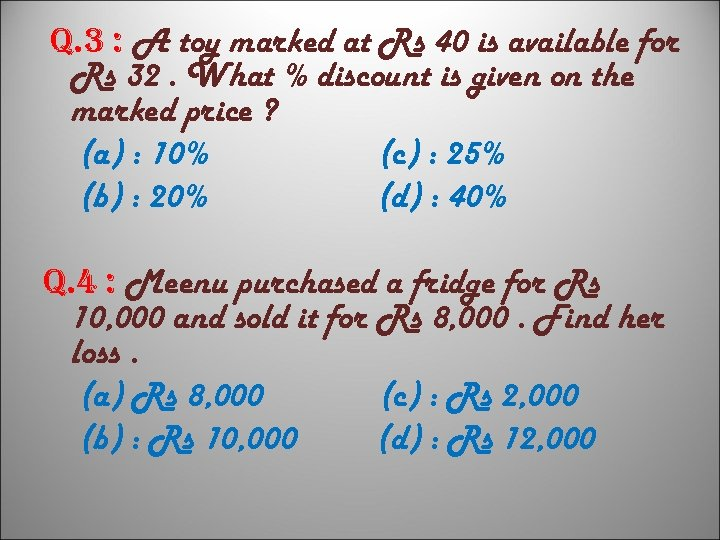q. 3 : A toy marked at Rs 40 is available for Rs 32. What % discount is given on the marked price ? (a) : 10% (c) : 25% (b) : 20% (d) : 40% q. 4 : Meenu purchased a fridge for Rs 10, 000 and sold it for Rs 8, 000. Find her loss. (a) Rs 8, 000 (c) : Rs 2, 000 (b) : Rs 10, 000 (d) : Rs 12, 000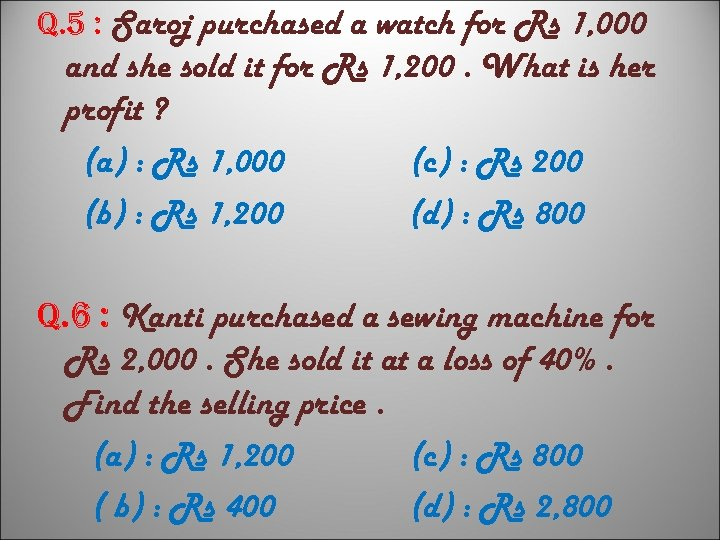q. 5 : Saroj purchased a watch for Rs 1, 000 and she sold it for Rs 1, 200. What is her profit ? (a) : Rs 1, 000 (c) : Rs 200 (b) : Rs 1, 200 (d) : Rs 800 q. 6 : Kanti purchased a sewing machine for Rs 2, 000. She sold it at a loss of 40%. Find the selling price. (a) : Rs 1, 200 (c) : Rs 800 ( b) : Rs 400 (d) : Rs 2, 800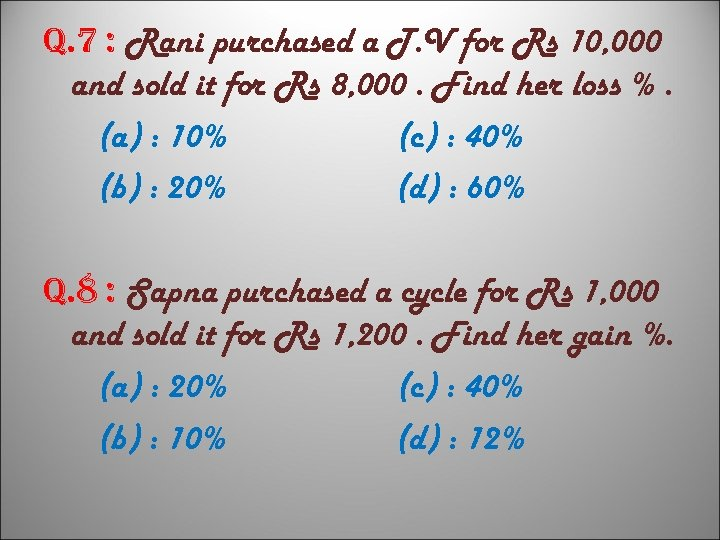q. 7 : Rani purchased a T. V for Rs 10, 000 and sold it for Rs 8, 000. Find her loss %. (a) : 10% (c) : 40% (b) : 20% (d) : 60% q. 8 : Sapna purchased a cycle for Rs 1, 000 and sold it for Rs 1, 200. Find her gain %. (a) : 20% (c) : 40% (b) : 10% (d) : 12%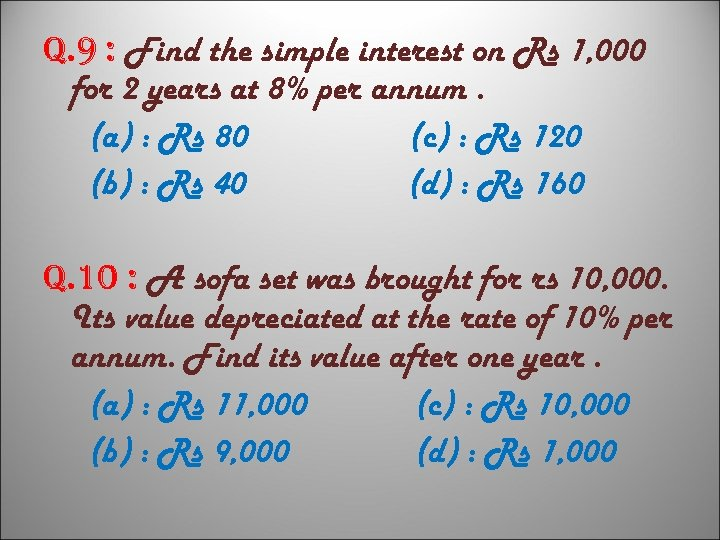q. 9 : Find the simple interest on Rs 1, 000 for 2 years at 8% per annum. (a) : Rs 80 (c) : Rs 120 (b) : Rs 40 (d) : Rs 160 q. 10 : A sofa set was brought for rs 10, 000. Its value depreciated at the rate of 10% per annum. Find its value after one year. (a) : Rs 11, 000 (c) : Rs 10, 000 (b) : Rs 9, 000 (d) : Rs 1, 000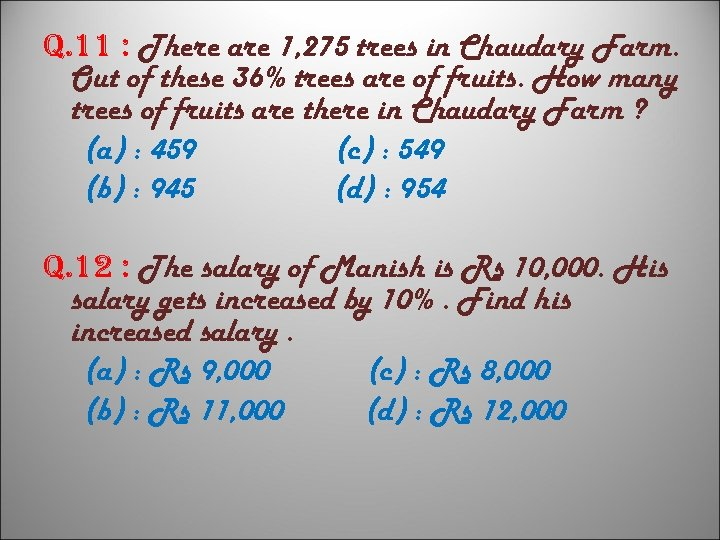q. 11 : There are 1, 275 trees in Chaudary Farm. Out of these 36% trees are of fruits. How many trees of fruits are there in Chaudary Farm ? (a) : 459 (c) : 549 (b) : 945 (d) : 954 q. 12 : The salary of Manish is Rs 10, 000. His salary gets increased by 10%. Find his increased salary. (a) : Rs 9, 000 (c) : Rs 8, 000 (b) : Rs 11, 000 (d) : Rs 12, 000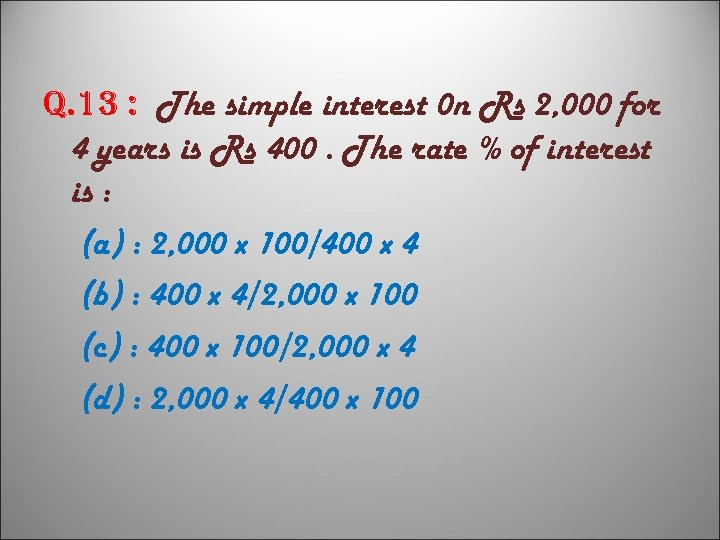q. 13 : The simple interest 0 n Rs 2, 000 for 4 years is Rs 400. The rate % of interest is : (a) : 2, 000 x 100/400 x 4 (b) : 400 x 4/2, 000 x 100 (c) : 400 x 100/2, 000 x 4 (d) : 2, 000 x 4/400 x 100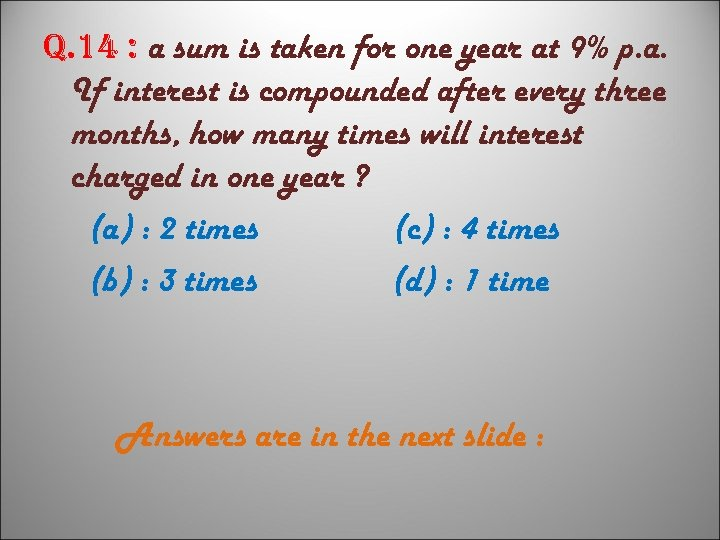q. 14 : a sum is taken for one year at 9% p. a. If interest is compounded after every three months, how many times will interest charged in one year ? (a) : 2 times (c) : 4 times (b) : 3 times (d) : 1 time Answers are in the next slide :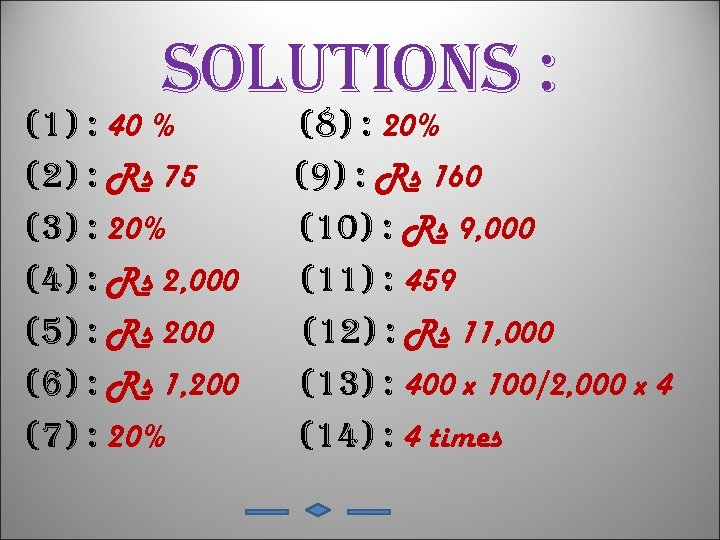solutions : (1) : 40 % (2) : Rs 75 (3) : 20% (4) : Rs 2, 000 (5) : Rs 200 (6) : Rs 1, 200 (7) : 20% (8) : 20% (9) : Rs 160 (10) : Rs 9, 000 (11) : 459 (12) : Rs 11, 000 (13) : 400 x 100/2, 000 x 4 (14) : 4 times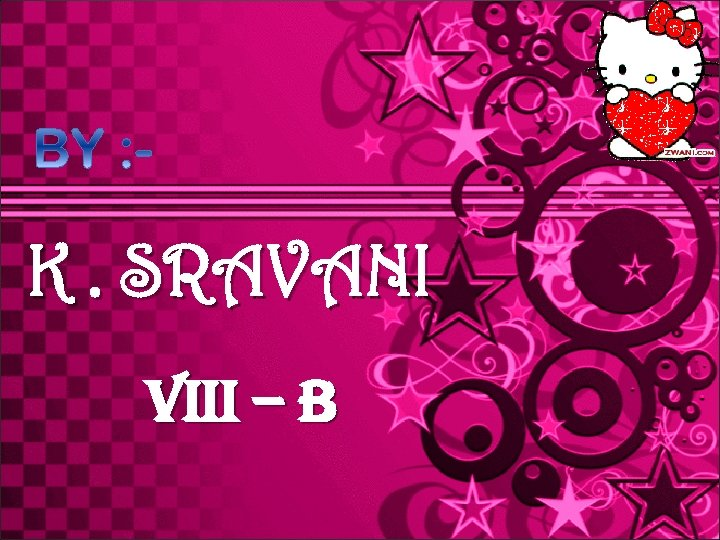K. SRAVANI Viii – B